# JP6163898B2 - Calculation device, calculation method, and calculation program - Google Patents

## Info

Publication number
JP6163898B2
JP6163898B2 JP2013122786A JP2013122786A JP6163898B2 JP 6163898 B2 JP6163898 B2 JP 6163898B2 JP 2013122786 A JP2013122786 A JP 2013122786A JP 2013122786 A JP2013122786 A JP 2013122786A JP 6163898 B2 JP6163898 B2 JP 6163898B2
Authority
JP
Japan
Prior art keywords
calculation
instruction
code
performance value
specific code
Prior art date
Legal status (The legal status is an assumption and is not a legal conclusion. Google has not performed a legal analysis and makes no representation as to the accuracy of the status listed.)
Active
Application number
JP2013122786A
Other languages
Japanese (ja)
Other versions
JP2014241031A (en
Inventor

Original Assignee

Priority date (The priority date is an assumption and is not a legal conclusion. Google has not performed a legal analysis and makes no representation as to the accuracy of the date listed.)
Filing date
Publication date
Application filed by 富士通株式会社 filed Critical 富士通株式会社
Priority to JP2013122786A priority Critical patent/JP6163898B2/en
Publication of JP2014241031A publication Critical patent/JP2014241031A/en
Application granted granted Critical
Publication of JP6163898B2 publication Critical patent/JP6163898B2/en
Active legal-status Critical Current
Anticipated expiration legal-status Critical

• 238000004364 calculation methods Methods 0.000 title claims description 614
• 238000004088 simulation Methods 0.000 claims description 179
• 238000003860 storage Methods 0.000 claims description 103
• 238000006243 chemical reactions Methods 0.000 claims description 22
• 230000000875 corresponding Effects 0.000 claims description 14
• 238000000034 methods Methods 0.000 description 70
• 238000010586 diagrams Methods 0.000 description 59
• 238000005457 optimization Methods 0.000 description 47
• 230000000576 supplementary Effects 0.000 description 26
• 230000001419 dependent Effects 0.000 description 20
• 280000233134 Hardware Resources companies 0.000 description 5
• 230000003111 delayed Effects 0.000 description 4
• 238000004458 analytical methods Methods 0.000 description 1
• 230000006399 behavior Effects 0.000 description 1
• 238000007796 conventional methods Methods 0.000 description 1
• 238000007689 inspection Methods 0.000 description 1
• 238000004519 manufacturing process Methods 0.000 description 1
• 230000003287 optical Effects 0.000 description 1
• 230000004044 response Effects 0.000 description 1
• 230000003068 static Effects 0.000 description 1

## Images

•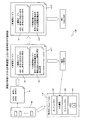••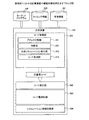•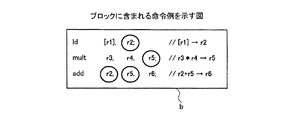•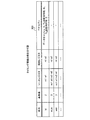•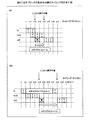•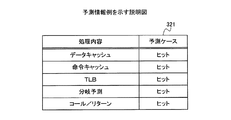•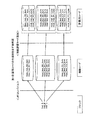•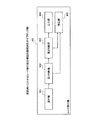•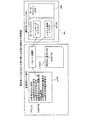••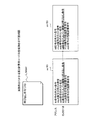•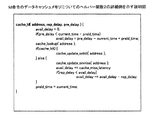•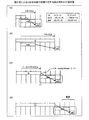•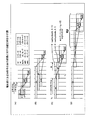•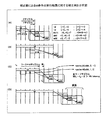•••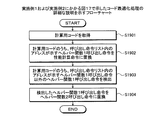•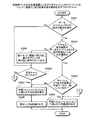•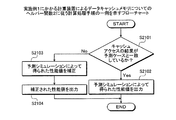•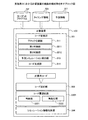•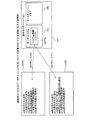•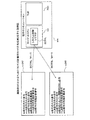•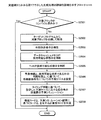•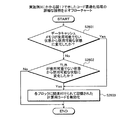•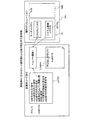•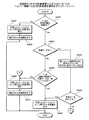•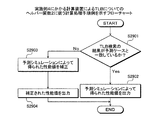•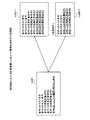•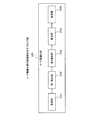•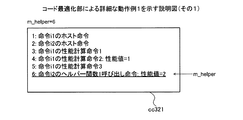•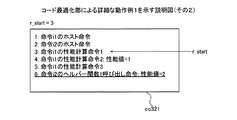•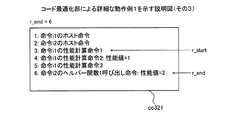•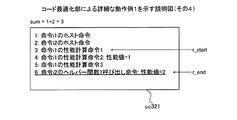•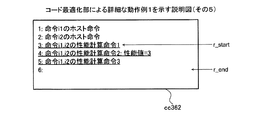•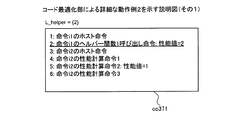•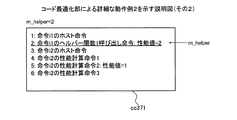•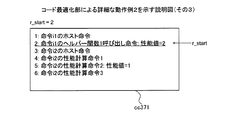•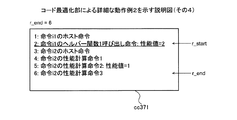•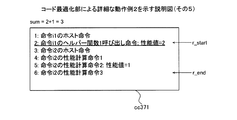•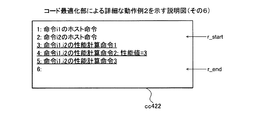•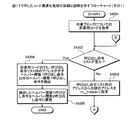•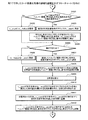## Classifications

• GPHYSICS
• G06COMPUTING; CALCULATING; COUNTING
• G06FELECTRIC DIGITAL DATA PROCESSING
• G06F8/00Arrangements for software engineering
• G06F8/40Transformation of program code
• G06F8/52Binary to binary
• GPHYSICS
• G06COMPUTING; CALCULATING; COUNTING
• G06FELECTRIC DIGITAL DATA PROCESSING
• G06F11/00Error detection; Error correction; Monitoring
• G06F11/30Monitoring
• G06F11/34Recording or statistical evaluation of computer activity, e.g. of down time, of input/output operation ; Recording or statistical evaluation of user activity, e.g. usability assessment
• G06F11/3457Performance evaluation by simulation
• GPHYSICS
• G06COMPUTING; CALCULATING; COUNTING
• G06FELECTRIC DIGITAL DATA PROCESSING
• G06F12/00Accessing, addressing or allocating within memory systems or architectures
• G06F12/08Addressing or allocation; Relocation in hierarchically structured memory systems, e.g. virtual memory systems
• G06F12/0802Addressing of a memory level in which the access to the desired data or data block requires associative addressing means, e.g. caches
• GPHYSICS
• G06COMPUTING; CALCULATING; COUNTING
• G06FELECTRIC DIGITAL DATA PROCESSING
• G06F12/00Accessing, addressing or allocating within memory systems or architectures
• G06F12/08Addressing or allocation; Relocation in hierarchically structured memory systems, e.g. virtual memory systems
• G06F12/0802Addressing of a memory level in which the access to the desired data or data block requires associative addressing means, e.g. caches
• G06F12/0888Addressing of a memory level in which the access to the desired data or data block requires associative addressing means, e.g. caches using selective caching, e.g. bypass
• GPHYSICS
• G06COMPUTING; CALCULATING; COUNTING
• G06FELECTRIC DIGITAL DATA PROCESSING
• G06F12/00Accessing, addressing or allocating within memory systems or architectures
• G06F12/08Addressing or allocation; Relocation in hierarchically structured memory systems, e.g. virtual memory systems
• G06F12/1027Address translation using associative or pseudo-associative address translation means, e.g. translation look-aside buffer [TLB]
• GPHYSICS
• G06COMPUTING; CALCULATING; COUNTING
• G06FELECTRIC DIGITAL DATA PROCESSING
• G06F8/00Arrangements for software engineering
• G06F8/40Transformation of program code
• G06F8/41Compilation
• G06F8/44Encoding
• G06F8/443Optimisation
• G06F8/4441Reducing the execution time required by the program code
• G06F8/4442Reducing the number of cache misses; Data prefetching
• GPHYSICS
• G06COMPUTING; CALCULATING; COUNTING
• G06FELECTRIC DIGITAL DATA PROCESSING
• G06F9/00Arrangements for program control, e.g. control units
• G06F9/06Arrangements for program control, e.g. control units using stored programs, i.e. using an internal store of processing equipment to receive or retain programs
• G06F9/44Arrangements for executing specific programs
• G06F9/455Emulation; Interpretation; Software simulation, e.g. virtualisation or emulation of application or operating system execution engines

## Description

The present invention relates to a calculation device, a calculation method, and a calculation program.

Conventionally, a CPU (Central Processing Unit) outputs an operation result of a cache memory accessible by the CPU during a simulation of an operation for executing the program, and the performance value of the program when the CPU executes the program is based on the operation result. (For example, refer to Patent Document 1 below).

JP 2001-249829 A

However, conventionally, each time an access instruction that instructs the CPU to access a storage area is executed during an operation simulation, the performance value of the program is calculated by referring to the operation result of the cache memory. Therefore, there is a problem that the amount of calculation for calculating the performance value of the access instruction increases.

In one aspect, an object of the present invention is to provide a calculation device, a calculation method, and a calculation program capable of reducing the amount of calculation.

According to an aspect of the present invention, the processor executes a program having a specific code that is executed a plurality of times and has a specific code having an access instruction that instructs the processor to access a storage area. When calculating the performance value of the program, if the specific code is a target for calculating the performance value, the attribute of the storage area at the time of execution of the access instruction in the simulation of the operation in which the processor executes the program, If it is determined whether the processor has an attribute indicating that the cache memory can be used in response to the instruction of the access instruction, and if the attribute is not an attribute indicating that the cache memory can be used, the cache memory Depending on the performance value of each instruction in the specific code when not using A calculation code for calculating the performance value of the specific code when the processor executes the specific code is generated without depending on the attribute, and generated when the specific code is newly subjected to performance value calculation. A calculation device, a calculation method, and a calculation program for executing the calculation code are proposed.

According to one embodiment of the present invention, the amount of calculation can be reduced.

FIG. 1 is an explanatory diagram of an example of an operation performed by the computing device according to the embodiment. FIG. 2 is a block diagram of a hardware configuration example of the computing device according to the embodiment. FIG. 3 is a block diagram of a functional configuration example of the computing apparatus according to the first embodiment. FIG. 4 is a diagram illustrating an example of instructions included in a block. FIG. 5 is a diagram illustrating an example of timing information. FIG. 6 is a diagram illustrating an example of execution timing of each instruction in the block illustrated in FIG. FIG. 7 is an explanatory diagram illustrating an example of prediction information. FIG. 8 is an explanatory diagram of an example of generating the first calculation code. FIG. 9 is a block diagram of an example of a functional configuration of the code execution unit according to the first embodiment. FIG. 10 is an explanatory diagram of an execution example by the code execution unit according to the first embodiment. FIG. 11 is an explanatory diagram of a generation example of the second calculation code according to the first embodiment. FIG. 12 is an explanatory diagram of a generation example of the second calculation code according to the second embodiment. FIG. 13 is an explanatory diagram showing a detailed example of the helper function 2 for the data cache memory of the ld instruction. FIG. 14 is an explanatory diagram illustrating a correction example for the execution result of the ld instruction by the correction unit. FIG. 15 is a diagram illustrating a correction example for the execution result of the ld instruction by the correction unit. FIG. 16 is a diagram illustrating a correction example for the execution result of the ld instruction by the correction unit. FIG. 17 is a flowchart of a calculation processing procedure example performed by the calculation apparatus according to the first and second embodiments. FIG. 18 is a flowchart of a detailed description of the generation process illustrated in FIG. 17 according to the first and second embodiments. FIG. 19 is a flowchart of a detailed description of the code optimization process illustrated in FIG. 17 according to the first and second embodiments. FIG. 20 is a flowchart of a calculation processing procedure example according to the helper function 1 for the data cache memory by the calculation apparatus according to the first embodiment. FIG. 21 is a flowchart of an example of a calculation processing procedure according to the helper function 2 for the data cache memory by the calculation apparatus according to the first embodiment. FIG. 22 is a block diagram of a functional configuration example of the computing device according to the third embodiment. FIG. 23 is an explanatory diagram of a calculation code generation example 1 for the data cache memory according to the third embodiment. FIG. 24 is an explanatory diagram of a calculation code generation example 2 for the TLB according to the third embodiment. FIG. 25 is a flowchart of a detailed description of the generation process depicted in FIG. 17 according to the third embodiment. FIG. 26 is a flowchart of a detailed description of the code optimization process depicted in FIG. 17 according to the third embodiment. FIG. 27 is an explanatory diagram of an execution example by the code execution unit according to the fourth embodiment. FIG. 28 is a flowchart of a calculation processing procedure example according to the helper function 1 for TLB by the calculation apparatus according to the fourth embodiment. FIG. 29 is a flowchart of a calculation processing procedure example according to the helper function 2 for the TLB by the calculation apparatus according to the fourth embodiment. FIG. 30 is an explanatory diagram of a code optimization example by the calculation apparatus according to the fifth embodiment. FIG. 31 is a block diagram illustrating a detailed example of the code optimization unit. FIG. 32 is an explanatory diagram (part 1) of a detailed operation example 1 by the code optimization unit. FIG. 33 is an explanatory diagram (part 2) of a detailed operation example 1 by the code optimization unit. FIG. 34 is an explanatory diagram (part 3) of a detailed operation example 1 by the code optimization unit. FIG. 35 is an explanatory diagram (part 4) of a detailed operation example 1 by the code optimization unit. FIG. 36 is an explanatory diagram (part 5) illustrating a detailed operation example 1 by the code optimization unit. FIG. 37 is an explanatory diagram (part 1) of a detailed operation example 2 by the code optimization unit. FIG. 38 is an explanatory diagram (part 2) of a detailed operation example 2 by the code optimization unit. FIG. 39 is an explanatory diagram (part 3) of a detailed operation example 2 by the code optimization unit. FIG. 40 is an explanatory diagram (part 4) of a detailed operation example 2 by the code optimization unit. FIG. 41 is an explanatory diagram (part 5) of a detailed operation example 2 by the code optimization unit. FIG. 42 is an explanatory diagram (part 6) of a detailed operation example 2 by the code optimization unit. FIG. 43 is a flowchart (part 1) showing a detailed description of the code optimization processing shown in FIG. FIG. 44 is a flowchart (part 2) showing a detailed description of the code optimization processing shown in FIG.

In the present embodiment, a function and performance simulation when the first processor to be evaluated executes the target program is executed by the second processor included in the computing device. As a method for converting the target program of the first processor into a code executable by the second processor when the function and performance are simulated by the second processor, for example, an interpreter method or a JIT (Just-in-Time) compiler method is used. is there. The computing device according to the present embodiment performs performance simulation by the JIT compiler method. The first processor is called a target CPU, and the second processor is called a host CPU.

Exemplary embodiments of a calculation device, a calculation method, and a calculation program according to the present invention will be described below in detail with reference to the accompanying drawings.

FIG. 1 is an explanatory diagram of an example of an operation performed by the computing device according to the embodiment. The calculation device 100 is a computer that calculates the performance value of the target program pgr when the target CPU 101 executes the target program pgr. Here, the performance value is the number of cycles and execution time. A processor included in the computing device 100 is referred to as a host CPU. Here, each code divided from the target program pgr is referred to as a block. The target program pgr has a block bt having an access instruction for instructing the target CPU 101 to access the storage area A in the memory 103 and executed by the target CPU 101 a plurality of times. The access command is, for example, an ld command or a st command. Here, for example, the block bt has an instruction i 1 and an instruction i 2, and the instruction i 2 is an access instruction that instructs the target CPU 101 to access the storage area A in the memory 103.

Further, the computing device 100 executes a simulation sim of an operation when the target CPU 101 executes the target program pgr. The operation simulation sim here is, for example, a simulation by giving the target program pgr to a model of a system having the target CPU 101 and hardware resources accessible by the target CPU 101. In the example of FIG. 1, examples of hardware resources include a cache memory 102 and a memory 103. The system model used in this embodiment is, for example, a behavior model that reproduces only the function of the system using a hardware description language or the like. Here, the execution timing of each block bt of the operation simulation sim is the same as the timing at which the calculation code cc11 for calculating the performance value of the block bt is executed. Here, for example, the target CPU 101 is an ARM (registered trademark) architecture CPU, and the host CPU included in the computing device 100 is an x86 architecture CPU, for example.

The calculation device 100 generates the calculation code cc11 when the block bt is a calculation target. The calculation code cc11 has a function code fc1 and a performance evaluation code pc1. The function code fc1 has a host instruction for each instruction in the block bt obtained by compiling the block bt. The performance evaluation code pc1 is an instruction for calculating the performance value of the block bt when the target CPU 101 executes the block bt based on the performance value of each instruction in the block bt based on the attribute of the cache memory 102 accessible by the target CPU 101. Have. Specifically, the performance evaluation code pc1 includes a performance calculation instruction that is the first instruction of the instruction i1 and a helper function 1 call instruction that is the second instruction of the instruction i2. The performance calculation instruction of the instruction i1 is an instruction that causes the target CPU 101 to add the performance value of the instruction i1 to the performance value of the block bt. The helper function 1 call instruction of the instruction i2 calls the helper function 1 that corrects the performance value of the instruction i2 according to the attribute of the storage area A when the instruction i2 of the operation simulation sim is executed and the operation result of the cache memory 102. It is an instruction to make. The helper function 1 call instruction of the instruction i2 is an instruction for adding the performance value output by the execution of the helper function 1 to the performance value of the block bt.

Then, the computing device 100 executes the calculation code cc11. Here, the function simulation is performed by the calculation device 100 executing the function code fc1 in the calculation code cc11. Further, the computer 100 executes the performance evaluation code pc1 in the calculation code cc11 to perform performance simulation. When the calculation apparatus 100 executes the helper function 1 call instruction of the instruction i2 in the calculation code cc11, the calculation apparatus 100 calls the helper function 1. Next, the computing device 100 executes the called helper function 1. According to the helper function 1, the computing device 100 determines whether or not the attribute of the storage area A at the time of execution of the instruction i2 in the operation simulation sim is an attribute indicating that the target CPU 101 can use the cache memory 102. . For example, when the target CPU 101 is an ARM processor, the attributes of the storage area A are TEX, B, and C bit values set in the page table entry for the storage area A. For example, when the values of the TEX, B, and C bits are all 1, the computing device 100 determines that the attribute of the storage area A is an attribute indicating that the cache memory 102 can be used. When any value of the TEX, B, and C bits is not 1, the computing device 100 determines that the attribute of the storage area A is not an attribute indicating that the cache memory 102 can be used.

When the computing device 100 determines that the attribute indicates that the cache memory 102 can be used, the performance value of the block bt is corrected by correcting the performance value based on the operation result of the cache memory 102 in the operation simulation sim. Add to. The operation result of the cache memory 102 is either a cache hit or a miss hit.

Next, when the computing device 100 determines that the cache memory 102 is not an attribute indicating that the cache memory 102 can be used, the computing device 100 corrects the performance value when the cache memory 102 is not used, and converts the corrected performance value into the performance value of the block bt. to add. When the block bt becomes a new performance value calculation target, the calculation device 100 executes the calculation code cc11.

If the computing device 100 determines that the attribute is not an attribute indicating that the cache memory 102 can be used, the computing device 100 generates a calculation code cc12. The calculation code cc12 is a code for calculating the performance value of the block bt when the target CPU 101 executes the block bt, regardless of the attribute, based on the performance value of each instruction in the block bt when the cache memory 102 is not used. is there. Specifically, the computing device 100 replaces the helper function 1 call instruction of the instruction i2 with a performance calculation instruction that adds the performance value of the instruction i2 when the cache memory 102 is not used to the performance value of the block bt. Thereby, the computing device 100 generates the calculation code cc12 having the performance evaluation code pc2 having the performance calculation instruction of the instruction i2. When the block bt becomes a new performance value calculation target, the calculation apparatus 100 executes the generated calculation code cc12. Thereby, it is possible to reduce the amount of calculation when the block bt is newly calculated.

(Hardware configuration example of the computing device 100)
FIG. 2 is a block diagram of a hardware configuration example of the computing device according to the embodiment. The computing device 100 includes a host CPU 201, a ROM (Read Only Memory) 202, a RAM 203, a disk drive 204, and a disk 205. The computing device 100 includes an I / F (Interface) 206, an input device 207, and an output device 208. Each unit is connected by a bus 200.

Here, the host CPU 201 governs overall control of the computing device 100. The ROM 202 stores a program such as a boot program. The RAM 203 is a storage unit used as a work area for the host CPU 201. The disk drive 204 controls reading / writing of data with respect to the disk 205 according to the control of the host CPU 201. The disk 205 stores data written under the control of the disk drive 204. Examples of the disk 205 include a magnetic disk and an optical disk.

The I / F 206 is connected to a network NET such as a LAN (Local Area Network), a WAN (Wide Area Network), and the Internet through a communication line, and is connected to other devices via the network NET. The I / F 206 controls a network NET and an internal interface, and controls data input / output from an external device. For example, a modem or a LAN adapter may be employed as the I / F 206.

The input device 207 is an interface for inputting various data by a user operation such as a keyboard, a mouse, and a touch panel. The input device 207 can also capture images and moving images from the camera. The input device 207 can also capture audio from a microphone. The output device 208 is an interface that outputs data according to an instruction from the host CPU 201. Examples of the output device 208 include a display and a printer.

The present embodiment will be described separately in the first to fifth embodiments. In the first embodiment, in the simulation of the operation of the block including the access instruction, when the access destination of the access instruction is unusable in the cache memory, the calculation code for calculating the performance value of the block on the assumption that the cache memory is not used is provided. Generate.

In the second embodiment, when the access instruction in the target block can use the cache memory, the calculation code for calculating the performance value of the block by correcting the performance value without depending on the attribute of the storage area by the operation of the cache memory Is generated.

In the third embodiment, the calculation code for calculating the performance value of the target block is executed based on the performance value of the access instruction when the cache memory is not used until the cache memory changes from the unusable state to the usable state. To do. In the third embodiment, the performance value of the target block is calculated based on the performance value of the access instruction when the TLB is not used until the TLB (Translation Lookaside Buffer) changes from the unusable state to the usable state. Run the calculation code.

In the fourth embodiment, when the operation mode of the target CPU is a privileged mode at the time of execution of an access instruction in the operation simulation, the performance value of the target block is calculated based on the performance value of each instruction of the target block when the TLB becomes a hit. Generate code for calculation.

In the fifth embodiment, when replacing the helper function 1 call instruction with the performance calculation instruction in the first or fourth embodiment, consecutive performance calculation instructions are aggregated into one performance calculation instruction.

Example 1
The memory address space includes an area where the data cache memory can be used and an area where the data cache memory cannot be used. For example, an area to which a device is mapped is an area where the data cache memory cannot be used. For this reason, the performance value of an instruction to access an area where the data cache memory cannot be used is constant. Therefore, in the first embodiment, when the access destination of the access instruction is unusable in the simulation of the operation of the block including the access instruction, the calculation code for calculating the performance value of the block on the premise that the cache memory is not used Is generated. As a result, the amount of calculation when the block becomes a target again can be reduced.

(Functional configuration example of the computing device 100 according to the first embodiment)
FIG. 3 is a block diagram of a functional configuration example of the computing apparatus according to the first embodiment. The computing device 100 includes a code conversion unit 301, a code execution unit 302, a code optimization unit 303, and a simulation information collection unit 304.

The processing from the code conversion unit 301 to the simulation information collection unit 304 is coded in a simulation program stored in a storage device such as the disk 205 accessible by the host CPU 201, for example. Then, the host CPU 201 reads the calculation program stored in the storage device and executes the process coded in the calculation program. Thereby, the processing from the code conversion unit 301 to the simulation information collection unit 304 is realized. Further, the processing results of the respective units are stored in a storage device such as the RAM 203 and the disk 205, for example.

The timing information 320, the target program pgr, and the prediction information 321 are acquired in advance and stored in a storage device such as the RAM 203 or the disk 205.

The code conversion unit 301 generates a calculation code that can calculate the performance value when the target block is executed by the target CPU 101 based on the performance value of each instruction of the target block. The code execution unit 302 calculates a performance value when the target block is executed by the target CPU 101 by executing the calculation code.

Specifically, the code conversion unit 301 includes a block division unit 311, a determination unit 312, a prediction simulation execution unit 313, and a code generation unit 314.

The block dividing unit 311 divides the target program pgr input to the computing device 100 into blocks based on a predetermined criterion. As for the division timing, for example, when the target block changes, a new target block may be divided, or the target program pgr may be divided into a plurality of blocks in advance. The block unit to be divided may be, for example, a basic block unit or an arbitrary predetermined code unit. A basic block unit is a group of instructions from a branch instruction to the next branch instruction.

FIG. 4 is a diagram illustrating an example of instructions included in a block. As shown in FIG. 4, a block b in the target program pgr has three instructions; (1) “ld [r1], r2” (load); (2) “multi r3, r4, r5” ( (Multiplication); (3) It is assumed that the instruction “add r2, r5, r6” (addition) is included and is executed in the pipeline of the target CPU 101 in the order of (1) to (3). Each instruction r1 to r6 represents a register (address). Hereinafter, even if the block is not the same as the block shown in FIG.

FIG. 5 is a diagram illustrating an example of timing information. In the timing information 320 shown in FIG. 5, for the ld instruction, the source register rs1 (r1) can be used by the first processing element (e1) and the destination register rd (r2) can be used by the second processing element (e2). Represents that. In the multit instruction, the first source register rs1 (r3) is the first processing element (e1), the second source register rs2 (r4) is the second processing element (e2), and the destination register rd (r5) is 3 The second processing element (e3) indicates that each can be used. In the add instruction, the first source register rs1 (r2), the second source register rs2 (r5) are used in the first processing element (e1), and the destination register rd (r6) is used in the second processing element (e2). Indicates that it is possible.

FIG. 6 is a diagram illustrating an example of execution timing of each instruction in the block illustrated in FIG. From the timing information 320 shown in FIG. 5, the timing at which each instruction is input into the pipeline is the timing t + 1 for the mul instruction and the timing t + 2 for the add instruction, where the start of execution of the ld instruction is timing t. Since the first source register (r2) and the second source register (r5) of the add instruction are used by the ld instruction and the multi instruction, the start of the add instruction is after timing t + 4 when the execution of the ld instruction and the multi instruction is completed. Thus, a waiting time for two cycles (stall for two cycles) occurs.

Therefore, as shown in FIG. 6A, when the block b shown in FIG. 4 is simulated, the execution time of the block b is the case where the cache memory operation result when the ld instruction is executed is a cache hit. It can be seen that there are 6 cycles. Here, the operation result of the target CPU 101 and the hardware resources when the externally dependent instruction is executed is referred to as the externally dependent instruction execution result. The externally dependent instruction is an instruction that, when executed by the target CPU 101, causes the target CPU 101 or the operation of the hardware resource accessible by the target CPU 101 to be one of a plurality of operations. For example, examples of the externally dependent instruction include an ld instruction, a st instruction, and a branch instruction. FIG. 6B shows an example of timing when the execution result of the ld instruction of the block b shown in FIG. 4 is a cache miss. If the result of the ld instruction is a cache miss, an arbitrary time that is considered sufficient for re-execution (here, 6 cycles) is set as a penalty in the timing information 320, so this penalty cycle is set as a delay time. Added. Therefore, the execution of the second processing element (e2) is delayed at the timing t + 7. The mul instruction to be executed next to the ld instruction is executed as it is without being affected by the delay. However, the add instruction is after the timing t + 8 when the execution of the ld instruction is completed, and wait time for 4 cycles (4 cycles). Stall) occurs.

Therefore, as shown in FIG. 6B, when the instruction execution of the block b shown in FIG. 4 is simulated, the execution time is 10 cycles when the execution result of the ld instruction is a cache miss. .

FIG. 7 is an explanatory diagram illustrating an example of prediction information. The prediction information 321 is information that defines a result that has a high probability of being generated among the operation results of the target CPU 101 and the hardware resources accessible by the target CPU 101 when the target CPU 101 executes an externally dependent instruction in the target program pgr. . This operation result is referred to as a prediction case.

Further, for example, hardware resources accessible by the target CPU 101 include an instruction cache memory, a data cache memory, and a TLB in the MMU. The instruction cache memory is a memory that temporarily stores frequently used instructions. The data cache memory is a memory that temporarily stores frequently used data. The TLB is a memory that temporarily stores frequently used conversion information. The conversion information is information for converting a logical address and a physical address. For example, the target CPU 101 uses a data cache memory, TLB, or the like when executing an access instruction to a storage area such as an ld instruction and an st instruction. For example, since there is an ld instruction in the block b shown in FIG. 4, for the ld instruction, the prediction case of the data cache is “cache hit”, and the prediction case of the TLB search is “TLB hit”.

The determination unit 312 determines whether or not the target block b has been compiled. For example, the determination unit 312 can determine the next block to be calculated according to the performance simulation execution result or the operation simulation sim execution result. More specifically, the determination unit 312 determines whether there is a calculation code associated with the target block b. If there is a calculation code associated with the target block b, the determination unit 312 determines that the target block b has been compiled. When there is no calculation code associated with the target block b, the determination unit 312 determines that the target block b has not been compiled.

When it is determined that the target block b has not been previously calculated, the prediction simulation execution unit 313 sets each prediction case for the externally dependent instruction included in the target block b based on the prediction information 321. Then, the prediction simulation execution unit 313 refers to the timing information 320 and simulates the progress of execution of each instruction in the block b based on the prediction case. Thereby, the prediction simulation execution unit 313 calculates the performance value of each instruction in the block b when the set prediction case is assumed.

The code generation unit 314 generates a first calculation code for calculating the performance value of the target block b when the target CPU 101 executes the target block b based on the prediction simulation result. The generated first calculation code is associated with the target block b and stored in a storage device such as the RAM 203 and the disk 205, for example.

FIG. 8 is an explanatory diagram of an example of generating the first calculation code. The code generation unit 314 generates a function code by compiling the target block b. Further, the code generation unit 314 generates a performance calculation instruction for adding the performance value of each instruction in the target block b obtained by the prediction simulation to the performance value of the target block b. In addition, the code generation unit 314 generates a helper function 1 call instruction for calling the helper function 1 for the externally dependent instruction. The helper function 1 call instruction is an instruction for calling the helper function 1 and adding the performance value output by the processing according to the helper function 1 to the performance value of the target block b. The process according to the helper function 1 according to the first embodiment is a process for correcting and outputting the performance value of the access instruction based on the attribute of the storage area of the access destination indicated by the access instruction and the operation of the cache memory.

For externally dependent instructions other than access instructions, the code generation unit 314 also corrects the performance value of the externally dependent instruction in the prediction case based on whether the execution result of the externally dependent instruction matches the prediction case. A helper function for instructing processing to be performed is generated. Then, the code generation unit 314 generates a first calculation code in which a performance evaluation code including each generated performance calculation instruction and helper function 1 call instruction is added to the function code. The generated first calculation code is stored in a storage device such as the RAM 203 or the disk 205 in association with the target block b. The code execution unit 302 executes a performance simulation for calculating the performance value of the target block b by executing the first calculation code.

FIG. 9 is a block diagram of an example of a functional configuration of the code execution unit according to the first embodiment. The code execution unit 302 includes an execution unit 901, a first determination unit 902, a second determination unit 903, an output unit 904, and a correction unit 905. The processes of the first determination unit 902, the second determination unit 903, the output unit 904, and the correction unit 905 are coded in the helper function 1 stored in a storage device such as the disk 205 accessible by the host CPU 201. The Then, the host CPU 201 reads the helper function 1 stored in the storage device, and executes the process coded in the helper function 1. Thereby, the process of the 1st judgment part 902, the 2nd judgment part 903, the output part 904, and the correction | amendment part 905 is implement | achieved. Further, the processing results of the respective units are stored in a storage device such as the RAM 203 and the disk 205, for example.

FIG. 10 is an explanatory diagram of an execution example by the code execution unit according to the first embodiment. For example, the code execution unit 302 sequentially executes instructions included in the first calculation code cc101. For example, when the execution unit 901 executes the helper function 1 call instruction for the data cache memory 1001 of the ld instruction, the execution unit 901 calls the helper function 1 and executes the process instructed by the helper function 1. Thereby, the process from the 1st judgment part 902 to the correction | amendment part 905 is performed. In addition, the data cache memory 1001 is taken as an example of the cache memory, but the same processing is performed even with an instruction cache memory.

In the example of FIG. 10, the first determination unit 902 determines whether or not the cache memory is usable when the target block b is executed in the simulation sim of the operation in which the target CPU 101 executes the target program pgr. For example, when the target CPU 101 is an ARM processor, the first determination unit 902 detects the value of the C bit of the system control register SCTRL in the operation at the time of execution of the target block b in the operation simulation sim. Then, the first determination unit 902 determines whether the value of the C bit of the system control register SCTRL is 0 or 1. The first determination unit 902 determines that the data cache memory 1001 is not usable when the value of the C bit of the system control register SCTRL is 0. If the value of the C bit of the system control register SCTRL is 1, the first determination unit 902 determines that the data cache memory 1001 is in a usable state.

When it is determined that the data cache memory 1001 is in a usable state, the second determination unit 903 determines the attribute of the access destination when executing the access instruction in the operation simulation sim. Specifically, the second determination unit 903 determines whether the attribute indicates that the data cache memory 1001 can be used. The second determination unit 903 detects the values of the TEX, B, and C bits set in the page table entry for the access destination when the access instruction is executed in the operation simulation sim as the access destination attribute. For example, when the target CPU 101 is an ARM processor, the second determination unit 903 uses the values of the TEX, B, and C bits set in the page table entry in the TLB for the access destination when executing the ld instruction in the operation simulation sim. To detect.

Then, when the detected TEX, B, and C bit values are all 1, the second determination unit 903 determines that the access destination attribute is an attribute indicating that the data cache memory 1001 can be used. In addition, when any value of the detected TEX, B, and C bits is not 1, the second determination unit 903 determines that the access destination attribute is not an attribute indicating that the data cache memory 1001 can be used. .

If it is determined that the data cache memory 1001 cannot be used, the output unit 904 outputs the address of the storage area where the caller's helper function 1 call instruction is stored to the call instruction list L_helper.

When it is determined that the attribute does not indicate that the data cache memory 1001 can be used, the correction unit 905 determines that the performance value obtained by the prediction simulation is “miss hit” when the prediction case is “cache hit”. Correct it so that it becomes a performance value. Then, the correction unit 905 outputs the corrected performance value. If it is determined that the attribute does not indicate that the data cache memory 1001 can be used, the correction unit 905 corrects the performance value of the access instruction obtained by the prediction simulation when the prediction case is “mishit”. Output without. In the above example, since the prediction case is “cache hit”, the correction unit 905 adds the penalty value included in the timing information 320 to the performance value of the ld instruction, for example.

When it is determined that the attribute indicates that the data cache memory 1001 can be used, the correction unit 905 determines whether the operation of the data cache memory 1001 in the operation simulation sim matches the prediction case. When the correction unit 905 matches the prediction case, the correction unit 905 outputs the performance value without correcting the performance value obtained by the prediction simulation. When the correction unit 905 does not match the prediction case, the correction unit 905 corrects the performance value obtained by the prediction simulation and outputs the performance value. Here, since the prediction case is “cache hit”, the correction unit 905 determines whether or not the operation result of the data cache memory 1001 in the operation simulation sim is “cache miss”. If it is “cache miss”, the correction unit 905 adds the penalty value in the timing information 320 to the performance value of the ld instruction. A detailed example of the correction processing by the correction unit 905 will be described in the second embodiment.

If it is determined that the data cache memory 1001 cannot be used, the code optimization unit 303 generates a second calculation code. The second calculation code determines the performance value of the target block b when the host CPU 201 executes the target block b according to the performance value of each instruction in the target block b when the data cache memory 1001 is not used. It is a code to calculate without depending on.

FIG. 11 is an explanatory diagram of a generation example of the second calculation code according to the first embodiment. Specifically, the code optimization unit 303 adds the performance value of the ld instruction in the case of “cache miss” to the helper function 1 call instruction indicated by the address in the call instruction list L_helper in the first calculation code cc101. Replace with the performance calculation instruction to be executed. Thereby, the code optimization unit 303 generates the second calculation code cc112. The generated second calculation code cc112 is stored in a storage device such as the RAM 203 or the disk 205 in association with the target block b. Further, the first calculation code cc101 previously associated with the target block b is deleted when the second calculation code cc112 is newly associated with the target block b.

Further, when the block b becomes the target block b, the determination unit 312 determines that the target block b has been previously calculated. The code execution unit 302 executes a performance simulation for calculating the performance value of the target block b by executing the second calculation code cc112. As described above, the processes from the first determination unit 902 to the correction unit 905 included in the code execution unit 302 are coded in the helper function 1. Therefore, when the second calculation code cc112 in which the helper function 1 call instruction is not coded is executed, the processing from the first determination unit 902 to the correction unit 905 is not executed. Thereby, it is possible to speed up the calculation of the performance value when the target block b including the access instruction is newly calculated.

Further, the simulation information collection unit 304 collects the calculation result of the performance value of each block b, and calculates the performance value of the target program pgr when the target CPU 101 executes the target program pgr.

(Example 2)
In the second embodiment, when the access instruction in the block b can use the cache memory, the performance value of the block b is calculated by correcting the performance value without depending on the attribute of the storage area by the operation of the cache memory. Generate code. Thereby, when the block b is newly calculated, since the attribute of the storage area is not determined, the amount of calculation of the performance value can be reduced. In the second embodiment, detailed description of the same functions and configurations as those described in the first embodiment is omitted. In the second embodiment, the data cache memory 1001 is exemplified as in the first embodiment, but an instruction cache memory may be used.

First, when it is determined that the data cache memory 1001 can be used, the code optimization unit 303 generates a second calculation code. The second calculation code is a code that can be executed by the host CPU 201 and uses the performance value of the target block b as an attribute of the storage area according to the performance value of each instruction in the target code when the data cache memory 1001 is used. It is a code that calculates without depending on it. The generated second calculation code is stored in a storage device such as the RAM 203 or the disk 205 in association with the target block b. Also, the first calculation code previously associated with the target block b is deleted when the second calculation code is newly associated with the target block b.

FIG. 12 is an explanatory diagram of a generation example of the second calculation code according to the second embodiment. Specifically, the code optimization unit 303 detects a helper function 1 call instruction other than the helper function 1 call instruction indicated by the address in the call instruction list L_helper among the helper function 1 call instructions in the first calculation code cc101. . Then, the code optimizing unit 303 generates the second calculation code cc123 by replacing the detected helper function 1 call instruction with the helper function 2 call instruction. The helper function 2 call instruction is an instruction for calling the helper function 2 and adding the performance value output by the processing according to the helper function 2 to the performance value of the target block b. Here, in the second embodiment, in order to facilitate understanding, the first calculation code cc101 will be described with only the helper function 1 call instruction for the data cache as the helper function 1 call instruction. The helper function 2 does not include an instruction for instructing the process of detecting the access destination storage area attribute of the access instruction included in the helper function 1 and the process of determining whether or not the data cache memory 1001 is usable. It is a function. Specifically, the processing of the correction unit 905 is coded in the helper function 2.

As a result, when the target block b is newly calculated, the process of determining the access destination attribute for the access instruction determined to be an attribute indicating that the data cache memory 1001 can be used is not performed. . Therefore, the amount of calculation of the performance value can be reduced, and the calculation of the performance value of the target program pgr can be speeded up.

FIG. 13 is an explanatory diagram showing a detailed example of the helper function 2 for the data cache memory of the ld instruction. The second calculation code cc123 incorporates a helper function 2 “cache_ld (address, rep_delay, pre_delay)” call instruction.

“Rep_delay” of the helper function 2 is a time (grace time) that is not processed as a delay time out of the penalty time until execution of the next instruction using the return value of the load (ld) instruction. “Pre_delay” is a delay time received from the previous instruction. “−1” indicates that there is no delay in the previous instruction. “Rep_delay” and “pre_delay” are time information obtained from the result of the static analysis process of the performance simulation result obtained by the prediction simulation execution unit 313 and the timing information 320.

In the example illustrated in FIG. 13, when the difference between the current timing current_time and the execution timing preld_time of the previous ld instruction exceeds the delay time of the previous ld instruction pre_delay, The effective delay time “avail_delay” is obtained by adjusting the delay time “pre_delay” by the time between the execution timing “preld_time” of the previous ld instruction and the current timing “current_time”.

Next, when the operation result of the data cache memory 1001 is “cache miss”, the correction unit 905 adds a penalty time cache_miss_latency at the time of a cache miss to the effective delay time “avail_delay” to obtain a grace period. The performance value of the ld instruction is corrected based on rep_delay.

14 to 16 show examples of correction for the execution result of the ld instruction by the correction unit. FIG. 14 is an explanatory diagram illustrating a correction example for the execution result of the ld instruction by the correction unit. FIG. 14 illustrates a correction example in the case where one cache process occurs and one cache miss occurs.

In the example of FIG. 14, the following three-instruction simulation is executed.
“Ld [r1], r2: [r1] → r2;
multit r3, r4, r5: r3 * r4 → r5;
add r2, r5, r6: r2 + r5 → r6 "

FIG. 14A shows a chart example of instruction execution timing when the prediction case is “cache hit”. In this prediction case, a 2-cycle stall has occurred in the add instruction that is executed third. FIG. 14B shows a chart example of instruction execution timing in the case of “cache miss” different from the prediction case. In an execution result different from this prediction case, if the execution result of the ld instruction is a cache miss, a delay of a penalty cycle (6 cycles) occurs. Therefore, the multi instruction is executed without being affected by the delay, but the execution of the add instruction is delayed by four cycles because it waits for the completion of the ld instruction. FIG. 14C is a diagram illustrating an example of a command execution timing chart after correction by the correction unit 905.

Since the execution result of the ld instruction is a cache miss (execution result different from the prediction case), the correction unit 905 has a predetermined cache miss penalty time (6 cycles) in the remaining execution time (2-1 = 1 cycle). Is added to obtain an effective delay time (7 cycles). The effective delay time is the maximum delay time. Further, the correction unit 905 obtains the execution time (3 cycles) of the next multi instruction, determines that the execution time of the next instruction does not exceed the delay time, and subtracts the execution time of the next instruction from the effective delay time. The time (7-3 = 4 cycles) is defined as the execution time (delay time) in which the delay of the ld instruction occurs. The correction unit 905 sets a time (3 cycles) obtained by subtracting the above-described delay time from the effective delay time as a grace period. The grace time is the time when the delay as a penalty is graced. The correction unit 905 returns a grace period rep_delay = 3 and a previous instruction delay time pre_delay = −1 (no delay) with the helper function 2cache_ld (address, rep_delay, pre_delay).

With this correction, the execution time of the ld instruction becomes an execution time (1 + 4 = 5 cycles) obtained by adding the execution time and the delay time. From the execution completion timing t 1 , the execution time of the subsequent mul instruction and add instruction Calculated. That is, the execution time (3 cycles) of each of the mul instruction and the add instruction obtained from the processing result (prediction simulation result based on the prediction result) of the prediction simulation execution unit 313 in the corrected execution time (5 cycles) of the ld instruction The execution time (number of cycles) of this block b can be obtained by simply adding (3 cycles).

As a result, a correction process is performed by adding or subtracting only the execution time of an instruction whose execution result is different from the prediction, and for other instructions, just adding the execution time obtained during the simulation based on the prediction result, The number of simulation execution cycles when a cache miss occurs can be obtained with high accuracy.

FIG. 14D is a diagram illustrating the magnitude of an error when the number of cycles at the time of a cache miss according to the conventional technique is obtained by simple addition for comparison with the processing of the computing device 100. In the case of FIG. 14D, since the delay time of the ld instruction is added as it is, an error is actually caused due to a shift in execution timing of the mul instruction that is completed during execution of the ld instruction. I understand that.

FIG. 15 is a diagram illustrating a correction example for the execution result of the ld instruction by the correction unit. FIG. 15 illustrates an example of correction when two cache misses occur when two cache processes are executed. In the example of FIG. 15, the following five-instruction prediction simulation is executed.
“Ld [r1], r2: [r1] → r2;
ld [r3], r4: [r3] → r4;
multit r5, r6, r7: r5 * r6 → r7;
add r2, r4, r2: r2 + r4 → r2;
add r2, r7, r2: r2 + r7 → r2 ”

FIG. 15A shows a chart example of instruction execution timing when the prediction result in two cache processes is “cache hit”. In this prediction case, it is assumed that two ld instructions are executed after two cycles (one normal cycle + one added cycle). FIG. 15B shows a chart example of instruction execution timing in the case of “cache miss” in which both of the two cache processes are different from the prediction result. In the case of this misprediction, there is a cache miss in each of the two ld instructions, resulting in a penalty cycle (6 cycles) delay. However, the delay times of the two ld instructions overlap, and the mul instruction is also executed without being affected by the delay, and execution of the two add instructions is delayed until the completion of the second ld instruction. . FIG. 15C shows an example of an instruction execution timing chart after correction by the correction unit 905.

As described with reference to FIG. 14, the correction unit 905 corrects the delay time of the first ld instruction at timing t 0 and returns the helper function 2cache_ld (addr, 3, −1). Then, at the present time t 1, the correction unit 905, since the second execution result of the ld instruction is a cache miss (the prediction result miss), the penalty cycles remaining execution time of the ld instruction (6) To make the effective delay time (1 + 6 = 7 cycles).

The correcting unit 905 subtracts the delay time consumed from the effective delay time until the current timing t 1 (<current timing t 1 −previous instruction execution timing t 0 > −set interval) from the current timing t 1. The excess effective delay time is obtained (7- (6-2) = 3 cycles), and the excess effective delay time is set as the execution time of the second ld instruction. Further, the correction unit 905 subtracts the original execution time from the excess effective delay time (3-1 = 2 cycles) to obtain the delay time of the previous instruction. The correction unit 905, the effective delay time by subtracting the sum of the effective delay time has been exceeded by the delay time and the present time t 1 consumed up to the current time t 1 (7- (3 + 3 ) = 1 cycle), It is a grace period.

The correction unit 905 corrects the delay time of the second ld instruction at timing t 1 and then returns the helper function 2cache_ld (addr, 2, 1). By this correction, the timing at which the correction value (3 cycles) is added to the current timing t 1 becomes the timing of completion of execution of the ld instruction, and the execution time of the subsequent mul instruction and add instruction is added from that timing. Become.

FIG. 15D shows the magnitude of error when the number of cycles at the time of a cache miss according to the prior art is obtained by simple addition for comparison with the processing of the computing device 100. In the case of FIG. 15D, it can be seen that a large error (8 cycles) occurs because the delay time based on the penalty given to each of the two ld instructions is added as it is. Even in the processing of the correction unit 905 shown in FIG. 15C, there is an error (one cycle) as compared with the case of correctly simulating as shown in FIG. 15B, but the accuracy is much higher than in the conventional method. It can be seen that

FIG. 16 is a diagram illustrating a correction example for the execution result of the ld instruction by the correction unit. A correction example when one cache miss occurs in the case where two cache processes are executed will be described. In the example of FIG. 16, the same five-instruction simulation as that of the explanatory example shown in FIG. 15 is executed.

FIG. 16A shows a chart example of instruction execution timing when the prediction result in the two cache processes is “cache hit”. In this prediction case, as in the case of FIG. 15A, it is assumed that two ld instructions are executed after two cycles (one normal cycle + one added cycle). FIG. 16B is a chart example of instruction execution timing when the first ld instruction is a “cache miss” different from the prediction result and the result of the second ld instruction is the prediction result (cache hit). Show. In the case of this misprediction, a delay of penalty cycle (6 cycles) occurs in the first ld instruction. The second ld instruction and the multi instruction are executed without being affected by the delay. The execution of the two add instructions will be delayed until the completion of the first ld instruction. FIG. 16C shows an example of an instruction execution timing chart after correction by the correction unit 905.

As described with reference to FIG. 14, the correction unit 905 corrects the delay time of the first ld instruction at timing t 0 and returns the helper function 2cache_ld (addr, 3, −1). Then, at the present time t 1, the correction unit 905, since the second execution result of the ld instruction is a cache hit (prediction result), the time from the start of the ld instruction to the current time t 1 <t 1− t 0 − It is determined whether the set interval (6-0−2 = 4 cycles)> is longer than the execution time of this ld instruction (2 cycles). Since the time from the start of execution of the second ld instruction to the current timing t 1 is longer than the execution time (2 cycles) of the ld instruction, the correction unit 905 executes the next multi instruction at the current timing t 1. Timing.

Then, the correction unit 905 treats the time from the completion of execution of the second ld instruction to the current timing t1 (2 cycles) as the delay time for the next instruction, and sets the delay time pre_delay = 2 of the previous instruction. Also, the correction unit 905 subtracts the sum of the delay time consumed until the current timing t 1 and the effective delay time exceeded at the current timing t 1 from the effective delay time of the first ld instruction (7− ( 6 + 0) = 1 cycle), the grace period rep_delay = 1 is set, and the helper function 2cache_ld (addr, 1, 2) is returned.

FIG. 16D shows the magnitude of error when the number of cycles at the time of a cache miss according to the prior art is obtained by simple addition for comparison with the processing of the computing device 100. In the case of FIG. 16D, it can be seen that an error occurs because the delay time due to the penalty of the first ld instruction is added as it is.

(Example of calculation processing procedure by the computer 100 according to the first and second embodiments)
FIG. 17 is a flowchart of a calculation processing procedure example performed by the calculation apparatus according to the first and second embodiments. The computing device 100 determines whether or not the calculation of the performance value of the target program pgr has been completed (step S1701). When the calculation has not been completed (step S1701: No), the computing device 100 performs calculation code generation processing (step S1702).

Next, the computing device 100 executes the calculation code associated with the target block b (step S1703). Further, the computing device 100 executes a simulation sim of the operation for the target block b when the target CPU 101 executes the target block b (step S1704). When the execution result of the operation simulation sim for the target block b is used for the execution of the calculation code, the execution of the calculation code waits for the execution of the operation simulation sim for the target block b to end.

After step S1703 and step S1704, the computing device 100 performs calculation code optimization processing (step S1705), and returns to step S1701. In step S1701, when it complete | finishes (step S1701: Yes), the calculation apparatus 100 collects a calculation result (step S1706), and complete | finishes a series of processes. In step S1706, for example, the computing device 100 obtains the performance value of the target program pgr when the target CPU 101 is executed on the target program pgr by summing the performance values of the respective blocks b.

FIG. 18 is a flowchart of a detailed description of the generation process illustrated in FIG. 17 according to the first and second embodiments. The computing device 100 determines whether or not the target block b has been compiled (step S1801). Next, the calculation apparatus 100 can specify the target block b to be calculated based on the value of the PC (Program Counter) in the operation simulation sim. Further, the computing device 100 can identify the target block b based on the execution result of the function code included in the calculation code.

When the target block b has not been compiled (step S1801: No), the computing device 100 divides and acquires the target block b from the target program pgr (step S1802). Next, the computing device 100 detects an externally dependent instruction in the target block b (step S1803).

Then, the computing device 100 sets a prediction case for the externally dependent instruction based on the prediction information 321 (step S1804). Subsequently, the computing device 100 performs a prediction simulation of the performance value of each instruction in the set prediction case based on the timing information 320 (step S1805). Next, the computing device 100 generates a calculation code having a function code obtained by compiling the target block b and a code based on the prediction simulation result (step S1806), and ends a series of processes. The calculation code generated in step S1806 is, for example, a first calculation code.

In step S1801, if the target block b has been compiled (step S1801: Yes), a series of processing ends.

FIG. 19 is a flowchart of a detailed description of the code optimization process illustrated in FIG. 17 according to the first and second embodiments. The computing device 100 acquires a calculation code (step S1901). The computing device 100 replaces the helper function 1 call instruction indicated by the address in the call instruction list L_helper in the calculation code with a performance calculation instruction (step S1902). The computing device 100 detects a helper function 1 call instruction for the data cache memory 1001 other than the helper function 1 call instruction indicated by the address in the call instruction list L_helper in the calculation code (step S1903). The computing device 100 replaces the detected helper function 1 call instruction with the helper function 2 call instruction for the data cache memory 1001 (step S1904), and ends the series of processes.

FIG. 20 is a flowchart of a calculation processing procedure example according to the helper function 1 for the data cache memory by the calculation apparatus according to the first embodiment. The computing device 100 determines whether or not the data cache memory 1001 is usable (step S2001). When the data cache memory 1001 is in a usable state (step S2001: Yes), the computing device 100 determines whether the access destination attribute in the operation simulation sim is an attribute indicating that the data cache memory 1001 can be used. Judgment is made (step S2002).

When it is determined that the attribute is an attribute indicating that the data cache memory 1001 can be used (step S2002: Yes), the computing device 100 determines whether the cache access result matches the predicted case in the operation simulation sim. It is determined whether or not (step S2003). The result of the cache access is an operation result of the data cache memory 1001. When the cache access result matches the prediction case (step S2003: Yes), the computing device 100 outputs the performance value obtained by the prediction simulation (step S2004), and ends the series of processes. If the result of the cache access does not match the prediction case (step S2003: No), the computing device 100 proceeds to step S2007.

When it is determined that the attribute is not an attribute indicating that the data cache memory 1001 can be used (step S2002: No), the computing device 100 outputs the address of the current helper function 1 call instruction to the call instruction list L_helper (step S200). S2005). The computing device 100 determines whether or not the prediction case is a hit (step S2006). When the prediction case is a hit (step S2006: Yes), the computing device 100 corrects the performance value obtained by the prediction simulation (step S2007). Then, the computing device 100 outputs the corrected performance value (step S2008) and ends a series of processing. When the prediction case is not a hit (step S2006: No), the computing device 100 proceeds to step S2004.

In step S2001, when the data cache memory 1001 is not in a usable state (step S2001: No), the computing device 100 proceeds to step S2006.

FIG. 21 is a flowchart of an example of a calculation processing procedure according to the helper function 2 for the data cache memory by the calculation apparatus according to the first embodiment. The helper function 2 for the data cache memory 1001 omits the instructions for instructing the usable state of the data cache memory 1001 and the access destination attribute in the helper function 1 for the data cache memory 1001. It is a function.

First, the computing device 100 determines whether or not the cache access result matches the prediction case in the operation simulation sim (step S2101). If they match (step S2101: Yes), the computing device 100 outputs the performance value obtained by the prediction simulation (step S2102), and ends the series of processes.

When they do not match (step S2101: No), the computing device 100 corrects the performance value obtained by the prediction simulation (step S2103). The computing device 100 outputs the corrected performance value (step S2104), and ends the series of processes.

(Example 3)
During initialization of the target CPU 101, the cache memory is not usable. Therefore, when the initialization of the target CPU 101 is completed, the cache memory becomes usable. Therefore, in the third embodiment, for calculating the performance value of the target block b based on the performance value of the access instruction when the cache memory is not used until the cache memory changes from the unusable state to the usable state. Run the code. Thereby, it is possible to reduce the amount of calculation of the performance value of the target block b during the period in which the cache memory is not usable, and to speed up the calculation of the performance value of the target program pgr.

In the third embodiment, detailed description of the same functions and configurations as those described in the first or second embodiment is omitted. In the third embodiment, the data cache memory 1001 is exemplified as in the first and second embodiments. However, an instruction cache memory may be used.

Further, the TLB is not usable during the initialization of the target CPU 101. Therefore, when the initialization of the target CPU 101 is completed, the TLB is ready for use. Therefore, in the third embodiment, calculation code for calculating the performance value of the target block b based on the performance value of the access instruction when the TLB is not used until the TLB changes from the unusable state to the usable state. Run. As a result, it is possible to reduce the amount of calculation of the performance value of the target block b during the period in which the TLB is not usable, and to speed up the calculation of the performance value of the target program pgr.

(Functional configuration example of the computing device 100 according to the third embodiment)
FIG. 22 is a block diagram of a functional configuration example of the computing device according to the third embodiment. The computing device 100 includes a code conversion unit 301, a code execution unit 302, a code optimization unit 2201, and a simulation information collection unit 304.

The processing from the code conversion unit 301 to the simulation information collection unit 304 illustrated in FIG. 22 is coded in a calculation program stored in a storage device such as the disk 205 accessible by the host CPU 201, for example. Then, the host CPU 201 reads the calculation program stored in the storage device and executes the process coded in the calculation program. Thereby, the processing from the code conversion unit 301 to the simulation information collection unit 304 is realized. Further, the processing results of the respective units are stored in a storage device such as the RAM 203 and the disk 205, for example.

Specifically, the code conversion unit 301 includes a block division unit 311, a first determination unit 2211, a second determination unit 2212, a prediction simulation execution unit 313, and a code generation unit 314. The block dividing unit 311 has the same function as the block dividing unit 311 described in the first embodiment.

First, the first determination unit 2211 determines whether the target block b has been compiled. A specific determination method by the first determination unit 2211 is the same as the determination method by the determination unit 312 described in the first embodiment.

(Data cache memory)
FIG. 23 is an explanatory diagram of a calculation code generation example 1 for the data cache memory according to the third embodiment. When it is determined that the data has not been compiled, the second determination unit 2212 determines whether or not the data cache memory 1001 is in a usable state when the access instruction in the target block b in the operation simulation sim is executed. Here, the target block b is not executed in the operation simulation sim. Therefore, the second determination unit 2212 determines whether or not the data cache memory 1001 is usable when the access instruction in the target block b is executed in the operation simulation sim based on the state in the current operation simulation sim. .

It is determined whether or not the data cache memory 1001 is in a usable state. Specifically, the second determination unit 2212 detects the value of the C bit of the system control register SCTRL. The second determination unit 2212 determines that the data cache memory 1001 is not in a usable state when the value of the C bit of the system control register SCTRL is 0. The second determination unit 2212 determines that the data cache memory 1001 is usable when the value of the C bit of the system control register SCTRL is 1.

The prediction simulation execution unit 313 acquires and sets each prediction case for the externally dependent instruction included in the target block b from the prediction information 321. Further, the prediction simulation execution unit 313 sets a prediction case related to the data cache memory 1001 for the access instruction based on the determination result by the second determination unit 2212. For example, when the prediction simulation execution unit 313 determines that the data cache memory 1001 is not usable, the prediction simulation execution unit 313 sets the prediction case for the data cache memory 1001 when the access instruction is executed to “cache miss”. For example, if the prediction simulation execution unit 313 determines that the data cache memory 1001 is usable, the prediction simulation execution unit 313 sets the prediction case for the data cache memory 1001 when the access instruction is executed to “cache hit”.

Then, the prediction simulation execution unit 313 refers to the timing information 320 to simulate the progress of execution of each instruction in the block b when the set prediction case is assumed. Thereby, the prediction simulation execution part 313 calculates | requires the performance value of each instruction in the object block b when assuming the set prediction case.

When the code generation unit 314 determines that the data cache memory 1001 is not usable, the code generation unit 314 generates the calculation code cc232. The generated calculation code cc232 is associated with the target block b and stored in a storage device such as the RAM 203 or the disk 205. The calculation code cc232 is a code for calculating the performance value of the target block b regardless of the state of the data cache memory 1001 based on the performance value of each instruction in the target block b when the data cache memory 1001 is not used. As shown in FIG. 23, when the data cache memory 1001 is not usable, the calculation code cc232 adds the performance value when the data cache memory 1001 is not used to the performance value of the target block b. Has calculation instructions. The code execution unit 302 executes the generated calculation code cc232. Thereby, the amount of calculation can be reduced.

When it is determined that the data cache memory 1001 is not usable, the code optimization unit 2201 invalidates all the calculation codes after the code execution unit 302 executes the calculation code cc232 associated with the target block b. Judgment is made. Specifically, the code optimization unit 2201 includes a determination unit 2221 and an invalidation unit 2222.

When it is determined that the data cache memory 1001 is not usable, the determination unit 2221 determines whether or not the data cache memory 1001 is usable after the calculation code cc232 is executed. Based on the determination by the determination unit 2221, a change from a state where the data cache memory 1001 is not usable to a usable state is detected. The determination method by the determination unit 2221 is the same as the determination method by the second determination unit 2212.

When the determination unit 2221 determines that the data cache memory 1001 is not usable, the invalidation unit 2222 does not invalidate the calculation code cc232 for each block b. For example, when the determination unit 2221 determines that the data cache memory 1001 is not in a usable state and the target block b becomes a new performance value calculation target, the code execution unit 302 executes the calculation code cc232. As a result, during the period when the data cache memory 1001 is not usable, the performance value of the target block b is calculated by the calculation code cc232.

On the other hand, when the determination unit 2221 determines that the data cache memory 1001 is usable, the invalidation unit 2222 invalidates the calculation code cc232 for each block b. Specifically, the invalidating unit 2222 invalidates the calculation code cc232 by deleting the calculation code cc232 stored in association with each block b. Accordingly, the first determination unit 2211 determines that the target block b has not been compiled because the calculation code associated with the target block b that has been newly calculated has been invalidated by the invalidation unit 2222.

If it is determined that the target block b has not been compiled, the second determination unit 2212 determines whether the data cache memory 1001 is in a usable state. As described above, when it is determined that the data cache memory 1001 is in a usable state, the prediction simulation execution unit 313 sets the prediction case for the data cache memory 1001 when the access instruction is executed to “cache hit”. Set to. The prediction simulation execution unit 313 refers to the timing information 320 and simulates the progress of execution of each instruction in the block b when the set prediction case is assumed. Thereby, the prediction simulation execution part 313 calculates | requires the performance value of each instruction in the object block b when assuming the set prediction case.

When the second determination unit 2212 determines that the data cache memory 1001 is usable because the value of the C bit of the system control register SCTRL is 1, the code generation unit 314 stores the calculation code cc233. Generate. The calculation code cc233 is a code for calculating the performance value of the block b based on the performance value of each instruction in the target block b when the data cache memory 1001 is used. As shown in FIG. 23, the calculation code cc233 has a helper function call instruction of an ld instruction that calls a helper function that corrects the performance value of the ld instruction in accordance with the cache access result of the data cache memory 1001. For example, the calculation code cc 233 having the helper function 1 call instruction shown in the first embodiment may be generated, whereby the first and third embodiments can be combined.

Then, the code execution unit 302 executes the generated calculation code cc233. In addition, once the data cache memory 1001 changes from the unusable state to the usable state, the data cache memory 1001 does not change to the unusable state again. During initialization of the target CPU 101, the data cache memory 1001 becomes unusable. Therefore, when the initialization of the target CPU 101 is completed, the data cache memory 1001 becomes usable. When the second determination unit 2212 determines that the data cache memory 1001 is usable, the code optimization unit 2201 according to the third embodiment does not perform processing.

(TLB)
FIG. 24 is an explanatory diagram of a calculation code generation example 2 for the TLB according to the third embodiment. In the case of the TLB 2401 as well, in the same way as in the case of the data cache memory 1001, processing of each unit is performed. Therefore, the second determination unit 2212 determines whether the TLB 2401 is in a usable state. Specifically, the second determination unit 2212 detects the M-bit value of the system control register SCTRL. The second determination unit 2212 determines that the TLB 2401 is not usable when the value of the M bit of the system control register SCTRL is 0. The second determination unit 2212 determines that the TLB 2401 is in a usable state when the value of the M bit of the system control register SCTRL is 1.

Based on the prediction information 321, the prediction simulation execution unit 313 acquires and sets each prediction case for the externally dependent instruction included in the target block b. Further, the prediction simulation execution unit 313 sets a prediction case related to the data cache memory 1001 for the access instruction based on the determination result by the second determination unit 2212. For example, when it is determined that the TLB 2401 is not usable, the prediction simulation execution unit 313 sets the prediction case for the data cache memory 1001 when the access instruction is executed to “TLB unavailable”. For example, if the prediction simulation execution unit 313 determines that the TLB 2401 is in a usable state, the prediction simulation execution unit 313 sets the prediction case for the TLB 2401 when the access instruction is executed to “cache hit”.

Then, as described above, the prediction simulation execution unit 313 obtains the performance value of each instruction in the target block b when the set prediction case is assumed.

The code generation unit 314 generates the calculation code cc242 when the second determination unit 2212 determines that the TLB 2401 is not usable. The calculation code cc242 is a code for calculating the performance value of the target block b regardless of the state of the TLB 2401 based on the performance value of each instruction in the target block b when the data cache memory 1001 is not used. The code execution unit 302 executes the generated calculation code cc242. As shown in FIG. 24, when the TLB 2401 is not in a usable state, the calculation code cc242 has a performance calculation instruction of an ld instruction that adds the performance value when the TLB 2401 is not used to the performance value of the target block b.

Since the processing of the code execution unit 302 and the code optimization unit 2201 is the same as that of the data cache memory 1001, detailed description thereof is omitted.

In addition, when the second determination unit 2212 determines that the TLB 2401 is usable, the code generation unit 314 generates the calculation code cc243. The calculation code cc243 is a code for calculating the performance value of the target block b based on the performance value of each instruction in the target block b when the TLB 2401 is used. As shown in FIG. 24, the calculation code cc243 has a helper function call instruction of an ld instruction that calls a helper function that corrects the performance value of the ld instruction in accordance with the TLB2401 search result. For example, the calculation code cc243 having the helper function 1 call instruction shown in the fourth embodiment may be generated, and the third and fourth embodiments can be combined.

For ease of understanding, the TLB 2401 and the data cache memory 1001 have been described by distinguishing the generation examples of the calculation code cc243, but in actuality, they are represented by the same performance calculation instruction and the same helper function.

(Example of calculation processing procedure by the calculation apparatus 100 according to the third embodiment)
In the third embodiment, the same calculation processing procedure example as the calculation processing procedure example by the calculation apparatus according to the first and second embodiments will not be described, and different calculation processing procedures will be described in detail.

FIG. 25 is a flowchart of a detailed description of the generation process depicted in FIG. 17 according to the third embodiment. The computing device 100 determines whether or not the target block b has been compiled (step S2501). Next, the calculation apparatus 100 can specify the target block b to be calculated based on the PC value in the operation simulation sim. Further, the computing device 100 can identify the target block b based on the execution result of the function code included in the calculation code.

When the target block b has not been compiled (step S2501: No), the computing device 100 divides and acquires the target block b from the target program pgr (step S2502). The computing device 100 detects an externally dependent instruction in the target block b (step S2503).

The computing device 100 determines whether the data cache memory 1001 is usable in the operation simulation sim (step S2504). Specifically, the computing device 100 makes a determination based on the value of the C bit of the system control register SCTRL in the operation simulation sim. The computing device 100 determines whether the TLB 2401 is usable in the operation simulation sim (step S2505). Specifically, the computing device 100 makes a determination based on the value of the C bit of the system control register SCTRL in the operation simulation sim.

The computing device 100 sets a prediction case for the externally dependent instruction based on the prediction information 321 and the determination result as to whether or not it is usable (step S2506). The computing device 100 performs a prediction simulation of the performance value of each instruction in the set prediction case based on the timing information 320 (step S2507). The computing device 100 generates a calculation code having a function code obtained by compiling the target block b and a code based on the prediction simulation result (step S2508), and ends a series of processes. The calculation code generated in step S2508 is the first calculation code.

In step S2501, if the target block b has been compiled (step S2501: Yes), a series of processing ends.

FIG. 26 is a flowchart of a detailed description of the code optimization process depicted in FIG. 17 according to the third embodiment. The computing device 100 determines whether or not the data cache memory 1001 has changed from an unusable state to an usable state (step S2601). If not changed (step S2601: No), the computing device 100 determines whether or not the TLB 2401 has changed from an unusable state to an available state (step S2602). When changed in step S2602 (step S2602: Yes) or changed in step S2601 (step S2601: Yes), the computing device 100 invalidates the calculation code stored in association with each block b (step S2603), a series of processing ends. Specifically, the computing device 100 deletes the calculation code stored in association with each block b.

On the other hand, when it has not changed (Step S2602: No), computing device 100 ends a series of processings. Also, either step S2601 or step S2602 may be executed first. For example, if the calculation code is invalidated in step S2603, it is determined in step S2501 that the code has not been compiled, and a new calculation code for the target block b is generated.

Example 4
When the target CPU 101 is an ARM processor, the target CPU 101 has two operation modes: a first mode and a second mode in which the operation restriction of the target CPU 101 is smaller than that in the first mode. The first mode is referred to as a user mode, and the second mode is a privileged mode. There are two operation modes, a privilege mode and a user mode. All operation modes other than the user mode are called privileged modes. The privileged mode is an operation mode in which there are fewer restrictions on the operation of the processor than in the user mode. Therefore, in the privileged mode, it is possible to access the system area in which the kernel is stored. Access to the system area in the privileged mode is designed in advance to hit the TLB 2401. For example, in the TLB 2401, the system area is managed by one page table entry. The TLB 2401 is designed in advance so that the page table entry is not deleted from the TLB 2401 using a special flag for the system area. Thus, the access can be realized to hit the TLB 2401. Therefore, when the target CPU 101 executes an access instruction in the privileged mode, the system area is accessed, so that the operation of the TLB 2401 for the access instruction becomes “hit”.

Therefore, in the fourth embodiment, when the operation mode is the privileged mode when the access instruction is executed in the operation simulation sim, the performance value of the target block b is calculated from the performance value of each instruction of the target block b on the assumption that the TLB is hit. Generate code for calculation. As a result, when the target block b is newly calculated, the processing for determining whether or not the TLB is a hit is not performed. Therefore, the calculation amount when the target block b is newly calculated Can be reduced. In the fourth embodiment, detailed description of the same functions and configurations as those described in any of the first to third embodiments is omitted.

First, the code generation unit 314 generates a function code by compiling the target block b. Further, the code generation unit 314 generates a performance calculation instruction for adding the performance value of each instruction in the target block b obtained by the prediction simulation to the performance value of the target block b. In addition, the code generation unit 314 generates a helper function 1 call instruction for calling the helper function 1 for the externally dependent instruction. The helper function 1 call instruction is an instruction for calling the helper function 1 and adding the performance value output by the processing according to the helper function 1 to the performance value of the target block b. The process according to the helper function 1 according to the fourth embodiment is a process for correcting and outputting the performance value of the access instruction based on the operation mode of the target CPU 101 and the state of the TLB 2401 when the access instruction is executed.

For externally dependent instructions other than access instructions, the code generation unit 314 also corrects the performance value of the externally dependent instruction in the prediction case based on whether the execution result of the externally dependent instruction matches the prediction case. A helper function for instructing processing to be performed is generated. Then, the code generation unit 314 generates a first calculation code in which each generated performance calculation instruction and helper function 1 call instruction are added to the function code. The generated first calculation code is stored in a storage device such as the RAM 203 or the disk 205 in association with the target block b.

Next, the code execution unit 302 performs a performance simulation for calculating the performance value of the target block b by executing the first calculation code. Similar to the first exemplary embodiment, the code execution unit 302 includes an execution unit 901, a first determination unit 902, a second determination unit 903, an output unit 904, and a correction unit 905.

FIG. 27 is an explanatory diagram of an execution example by the code execution unit according to the fourth embodiment. For example, the code execution unit 302 sequentially executes instructions included in the first calculation code cc2701. Then, when the execution unit 901 executes the helper function 1 call instruction for the TLB 2401 of the ld instruction, the execution unit 901 calls the helper function 1 and executes the process instructed by the helper function 1. Thereby, the process from the 1st judgment part 902 to the correction | amendment part 905 is performed.

In the example of FIG. 27, the first determination unit 902 determines whether or not the TLB 2401 is valid in the operation at the time of execution of the target block b in the simulation sim of the operation in which the target CPU 101 executes the target program pgr. For example, when the target CPU 101 is an ARM processor, the first determination unit 902 detects the value of the M bit in the system control register SCTRL in the operation at the time of execution of the target block b in the operation simulation sim. Then, the first determination unit 902 determines whether the M-bit value of the system control register SCTRL is 0 or 1. The first determination unit 902 determines that the TLB 2401 is not usable when the M-bit value of the system control register SCTRL is 0, and if the M-bit value of the system control register SCTRL is 1, the TLB 2401 It is determined that it can be used.

When it is determined that the TLB 2401 is in a usable state, the second determination unit 903 determines whether or not the operation mode of the target CPU 101 when executing the access instruction in the target block b is the privileged mode in the operation simulation sim. to decide.

Specifically, when the target CPU 101 is an ARM processor, the second determination unit 903 detects the value of the M field of the CPSR register. The second determination unit 903 determines that the operation mode of the target CPU 101 is the user mode when the value of the M field of the CPSR register is “1000b”. Then, the second determination unit 903 determines that the operation mode of the target CPU 101 is the privilege mode when the value of the M field of the CPSR register is other than “1000b”.

When it is determined that the operation mode is the privileged mode, the output unit 904 outputs the address of the storage area where the caller's helper function 1 call instruction is stored to the call instruction list L_helper.

When it is determined that the operation mode is the privileged mode, the correction unit 905 outputs the access instruction without correcting the performance value if the prediction case is “hit”. When it is determined that the operation mode is the privileged mode, the correction unit 905 performs the performance of the access instruction when the operation of the TLB 2401 is “hit” based on the timing information 320 if the prediction case is “mishit”. Corrected to be a value and output.

When it is determined that the operation mode is the user mode, the correction unit 905 determines whether the operation result of the TLB 2401 in the operation simulation sim matches the prediction case if the prediction case is “mishit”. . When the correction unit 905 matches the prediction case, the correction unit 905 outputs the performance value of the access instruction without correction. When the correction unit 905 does not match the prediction case, the correction unit 905 corrects and outputs the performance value of the access instruction. In the above example, since the prediction case is “cache hit”, the correction unit 905 determines whether or not the operation of the TLB 2401 in the operation simulation sim is “miss hit”. Then, if “Mishit”, the correction unit 905 corrects the performance value by adding the penalty value in the timing information 320 to the performance value of the access instruction, and outputs the corrected value. A detailed example of the correction processing by the correction unit 905 will be described in the second embodiment.

Further, when it is determined that the TLB 2401 is invalid, the correction unit 905 adds the performance value of the access instruction to the performance value of the access instruction based on the timing information 320 to thereby obtain the performance value. Correct and output.

When it is determined that the operation mode is the privileged mode, the code optimization unit 2201 generates a second calculation code. The second calculation code uses the performance value of each instruction in the target block b when the operation of the TLB 2401 is “hit” and the performance value of the target block b when the host CPU 201 executes the target block b as the operation mode. It is a code to calculate without depending on. As described above, in the access to the system area, the operation of the TLB 2401 becomes “hit”, so that the process of correcting the performance value of the access instruction is not performed based on whether or not the operation of the TLB 2401 is “hit”. It's okay.

Specifically, the code optimization unit 2201 uses the performance value of the ld instruction in the case of “hit” for the helper function 1 call instruction indicated by the address in the call instruction list L_helper in the first calculation code cc2701. Replace with the performance calculation instruction to be added. Accordingly, the code optimization unit 2201 generates a second calculation code. The generated second calculation code is stored in a storage device such as the RAM 203 or the disk 205 in association with the target block b.

In addition, when the block b in which the second calculation code is generated becomes the target block b, the determination unit 312 determines that the second calculation code has been compiled because the second calculation code is associated with the target block b. And the code execution part 302 performs the performance simulation which calculates the performance value of the object block b by executing the 2nd calculation code. As described above, the processes from the first determination unit 902 to the correction unit 905 included in the code execution unit 302 are coded in the helper function 1. Therefore, the processing from the first determination unit 902 to the correction unit 905 is not executed when executing the second calculation code in which the helper function 1 call instruction is not coded.

Thereby, it is possible to speed up the calculation of the performance value when the target block b including the access instruction is newly calculated.

If it is determined that the operation mode is not the privilege mode, the code optimization unit 2201 generates a second calculation code. The second calculation code uses the performance value of the target block b when the host CPU 201 executes the target block b based on the performance value of each instruction in the target block b when the operation of the TLB 2401 is a prediction case. It is a code to calculate without.

(Example of calculation processing procedure by the calculation apparatus 100 according to the fourth embodiment)
In the fourth embodiment, the same calculation processing procedure example as that of the calculation processing procedure example by the calculation apparatus 100 according to the first and second embodiments will not be described, and different calculation processing procedures will be described in detail.

FIG. 28 is a flowchart of a calculation processing procedure example according to the helper function 1 for TLB by the calculation apparatus according to the fourth embodiment. The helper function 1 for the TLB 2401 is called when the host CPU 201 executes a helper function 1 call instruction, which is a performance simulation code for an access instruction such as a st instruction and an ld instruction.

The computing device 100 determines whether or not the TLB 2401 of the target CPU 101 is usable in the operation simulation sim (step S2801). As described above, when the target CPU 101 is an ARM processor, the computing device 100 determines whether the value of the M bit of the system control register SCTRL is 0 or 1 in the operation simulation sim. When the M bit value of the system control register SCTRL is 0, the computing device 100 determines that the TLB 2401 is not usable, and when the M bit value of the system control register SCTRL is 1, the TLB 2401 can be used. It is determined that the condition is not correct.

If the TLB 2401 is in a usable state (step S2801: YES), the computing device 100 determines whether or not the operation mode of the target CPU 101 is a privileged mode in the operation simulation sim (step S2802). For example, when the target CPU 101 is an ARM processor, the computing device 100 determines whether or not it is a privileged mode based on the value of the M bit of CPSR. The computing device 100 determines that the user mode is selected when the value of the M bit of the CPSR is 1000b. When the value of the M field of CPSR is other than 1000b, the computing device 100 determines that the mode is the privileged mode.

If the current mode is the privilege mode (step S2802: YES), the computing device 100 outputs the address of the current helper function call instruction to the call instruction list L_helper (step S2803). The computing device 100 determines whether or not the prediction case is a hit (step S2804). If the prediction case is a hit (step S2804: YES), the computing device 100 moves to step S2806. If the predicted case is not a hit (step S2804: NO), the computing device 100 proceeds to step S2809.

In the privilege mode, the system area is accessed by the ld instruction or the st instruction. As described above, for the access to the system area, the result of the TLB2401 inspection becomes a hit. Therefore, the performance value in the case of TLB2401 hit is output.

In step S2802, if the mode is not the privileged mode (step S2802: No), the computing device 100 determines whether the result of the TLB2401 search in the operation simulation sim matches the prediction case (step S2805). If they match (step S2805: YES), the computing device 100 outputs the performance value obtained by the prediction simulation (step S2806), and ends the series of processes. If they do not match (step S2805: NO), the computing device 100 corrects the performance value obtained by the prediction simulation (step S2809). In this case, the correction is made based on the TLB usable penalty value included in the timing information 320. Then, the computing device 100 outputs the corrected performance value (step S2810) and ends the series of processes.

In Step S2801, if the TLB 2401 is not usable (Step S2801: No), the computing device 100 corrects the performance value obtained by the prediction simulation (Step S2807). Here, the correction is made by the penalty value included in the timing information 320 that cannot be used for TLB. The computing device 100 outputs the corrected performance value (step S2808), and ends the series of processes.

FIG. 29 is a flowchart of a calculation processing procedure example according to the helper function 2 for the TLB by the calculation apparatus according to the fourth embodiment. The helper function 2 for the TLB 2401 is a function in which determination as to whether or not the helper function 1 is a privileged mode is omitted. The computing device 100 determines whether or not the result of the TLB2401 search in the operation simulation sim matches the prediction case (step S2901). If they match (step S2901: YES), the computing device 100 outputs the performance value obtained by the prediction simulation (step S2902), and ends the series of processes. If they do not match (step S2901: NO), the computing device 100 corrects the performance value obtained by the prediction simulation (step S2903). Then, the computing device 100 outputs the corrected performance value (step S2904) and ends the series of processes.

(Example 5)
In the fifth embodiment, when replacing the helper function 1 call instruction with the performance calculation instruction in the first or fourth embodiment, consecutive performance calculation instructions are aggregated into one performance calculation instruction. Thereby, it is possible to reduce the amount of calculation when the target block b is newly calculated. In the fifth embodiment, detailed description of the same functions and configurations as those described in any of the first to fourth embodiments is omitted.

FIG. 30 is an explanatory diagram of a code optimization example by the calculation apparatus according to the fifth embodiment. The code optimization unit 2201 according to the fifth embodiment generates a calculation code cc302-2 in which a helper function 1 call instruction in the calculation code cc301 and a continuous performance calculation instruction are replaced with a combined performance calculation instruction. To do. Compared with the calculation code cc302-1 when the helper function 1 call instruction is simply replaced with a performance calculation instruction, the amount of calculation can be reduced.

FIG. 31 is a block diagram illustrating a detailed example of the code optimization unit. The code optimization unit 2201 includes an acquisition unit 3101, a first detection unit 3102, a second detection unit 3103, a calculation unit 3104, and a replacement unit 3105.

First, an example in which a helper function 1 call instruction determined not to use the data cache memory 1001 described in the first embodiment is replaced with a performance calculation instruction will be described. The acquisition unit 3101 acquires the calculation code cc301 stored in association with the target block b.

The first detection unit 3102 detects a helper function 1 call instruction for an access instruction that is determined not to have an attribute indicating that the data cache memory 1001 can be used in the obtained calculation code cc301. The second detection unit 3103 performs performance calculation between the detected helper function 1 call instruction and the helper function 1 call instruction for the access instruction that is determined to be an attribute indicating that the data cache memory 1001 can be used. Detect instructions.

For example, the calculation unit 3104 calculates the total value of the performance value that the detected performance calculation instruction adds to the performance value of the target block b and the performance value of the access instruction when the data cache memory 1001 is not used.

The replacement unit 3105 converts the detected performance calculation instruction and the detected helper function 1 call instruction in the calculation code cc301 into a performance calculation instruction that adds the calculated total value to the performance value of the target block b. Replace.

Next, an example in which the helper function 1 call instruction that is determined to be the privileged mode as the operation mode of the target CPU 101 described in the fourth embodiment is replaced with a performance calculation instruction will be described. The acquisition unit 3101 acquires the calculation code cc301.

The first detection unit 3102 detects a helper function 1 call instruction for the access instruction determined to be in the privileged mode from the obtained calculation code cc301. The second detection unit 3103 detects a performance calculation instruction between the detected helper function 1 call instruction and the helper function 1 call instruction for the access instruction determined not to be in the privileged mode.

For example, the calculation unit 3104 calculates the performance value of the access instruction when the performance value that the detected performance calculation instruction adds to the performance value of the target block b and the conversion information between the logical address and the physical address are stored in the TLB. And the total value is calculated.

The replacement unit 3105 converts the detected performance calculation instruction and the detected helper function 1 call instruction in the calculation code cc301 into a performance calculation instruction that adds the calculated total value to the performance value of the target block b. Replace.

Next, a detailed operation example 1 by the code optimization unit 2201 will be described with reference to FIGS. 32 to 36, and a detailed operation example 2 by the code optimization unit 2201 will be described with reference to FIGS. 37 to 42.

32 to 36 are explanatory diagrams showing a detailed operation example 1 by the code optimization unit. First, the acquisition unit 3101 acquires the calculation code cc321. The number of performance calculation instructions for each instruction in the target block b is determined based on the configuration of the host CPU 201. In the example of the calculation code cc321, it is assumed that the performance calculation instruction for adding the performance value of each instruction to the target block b is realized by three instructions as follows.
Performance calculation instruction 1: Load performance value into register Performance calculation instruction 2: Add performance value to register Performance calculation instruction 3: Store register value into performance value

The first detection unit 3102 selects one address included in the call instruction list L_helper and sets the selected address as m_helper. Then, the first detection unit 3102 detects a helper function 1 call instruction indicated by m_helper. In the example of FIG. 32, in order to facilitate understanding, the order of instructions from the head is used as the instruction address. Since there are 6 in the call instruction list L_helper, the first detection unit 3102 detects the helper function 1 call instruction of the instruction i2.

For example, if the instruction coded immediately before the detected helper function 1 call instruction is a performance calculation instruction, the second detection unit 3103 sets the address indicating the performance calculation instruction as r_start. In addition, if the instruction coded immediately before the detected helper function 1 call instruction is not a performance calculation instruction, the second detection unit 3103 sets the address indicating the detected helper function 1 call instruction as r_start. In the example of FIG. 33, the address indicating the performance calculation instruction 1 of the instruction i1 is r_start.

Next, the second detection unit 3103 searches for the instruction subsequent to the helper function 1 call instruction of the detected instruction i2. Then, the second detection unit 3103 searches from the helper function 1 call instruction of the instruction i2 to the helper function 1 call instruction whose address is not registered in the call instruction list L_helper. The second detection unit 3103 indicates the instruction immediately before the unregistered helper function 1 call instruction among the instructions between the helper function 1 call instruction of the instruction i2 and the unregistered helper function 1 call instruction. The address is r_end. If there is no helper function 1 call instruction whose address is not registered in the call instruction list L_helper subsequent to the helper function 1 call instruction of the instruction i2, the second detection unit 3103 sets an address indicating the last instruction in the target block b. Let r_end. In the example of FIG. 34, r_end is an address indicating the helper 1 function call instruction of the instruction i2.

The calculation unit 3104 acquires a performance value that a performance calculation instruction in the instruction group from the instruction indicated by r_start to the instruction indicated by r_end adds to the performance value of the target block b. The calculation unit 3104 acquires a performance value that the helper function 1 call instruction in the instruction group adds to the performance value of the target block b. The performance value that the helper function 1 call instruction in the instruction group adds to the performance value of the target block b is, for example, the performance value of the access instruction when the data cache memory 1001 is not used. The performance value that the helper function 1 call instruction in the instruction group adds to the performance value of the target block b is, for example, the performance value of the access instruction when the TLB has conversion information. In the example of the helper function 2cache_ld (address, rep_delay, pre_delay) shown in FIG. 13, three arguments are passed to the helper function cache_ld. Therefore, when the host CPU 201 is an x86 architecture, specifically, the helper function 1 call instruction is
push pre_delay: put the third argument on the stack push rep_delay: put the second argument on the stack push addr: put the first argument on the stack call cache_ld: call the helper function 1 cache_ld. The calculation unit 3104 obtains the performance value of the helper function 1 call instruction by calculating the performance value when the data cache memory 1001 is not used with reference to pre_delay among the four instructions.

Then, the calculation unit 3104 calculates the total value of the acquired performance values. In the example of FIG. 35, since the performance value of the performance calculation instruction 2 of the instruction i1 is 1, and the performance value of the helper function 1 call instruction of the instruction i2 is 2, the total value sum is 3.

The replacement unit 3105 includes an instruction group in which a host instruction included in an instruction group from an instruction indicated by r_start to an instruction indicated by r_end and a performance calculation instruction for adding the total value sum to the performance value of the target block b are arranged in this order. create. As illustrated in FIG. 36, the replacement unit 3105 generates the calculation code cc362 by replacing the instruction group from the instruction indicated by r_start to the instruction indicated by r_end with the created instruction group. The replacement unit 3105 deletes m_helper from the call instruction list L_helper after the replacement is completed. The replacement unit 3105 corrects each address included in the call instruction list L_helper based on the calculation code cc321 before replacement and the calculation code cc362 after replacement. Next, the code optimization unit 2201 repeats the processing from the acquisition unit 3101 to the replacement unit 3105 until the calling instruction list L_helper becomes empty.

37 to 42 are explanatory diagrams showing a detailed operation example 2 by the code optimization unit. First, the acquisition unit 3101 acquires the calculation code cc371. The first detection unit 3102 selects one address included in the call instruction list L_helper and sets the selected address as m_helper. Then, the first detection unit 3102 detects a helper function 1 call instruction indicated by m_helper. In the example of FIG. 37, in order to facilitate understanding, the order of instructions from the top is used as an instruction address. Since there are 2 in the call instruction list L_helper, the first detection unit 3102 detects the helper function 1 call instruction of the instruction i1.

For example, if the instruction coded immediately before the detected helper function 1 call instruction is a performance calculation instruction, the second detection unit 3103 sets the address indicating the performance calculation instruction as r_start. In addition, if the instruction coded immediately before the detected helper function 1 call instruction is not a performance calculation instruction, the second detection unit 3103 sets an address indicating the detected helper function 1 call instruction as r_start. In the example of FIG. 39, the address indicating the helper function 1 call instruction of the instruction i1 is r_start.

Next, the second detection unit 3103 searches for the instruction subsequent to the helper function 1 call instruction of the detected instruction i2. The second detection unit 3103 searches from the helper function 1 call instruction of the instruction i2 to the helper function 1 call instruction whose address is not registered in the call instruction list L_helper. The second detection unit 3103 indicates the instruction immediately before the unregistered helper function 1 call instruction among the instructions between the helper function 1 call instruction of the instruction i2 and the unregistered helper function 1 call instruction. The address is r_end. If there is no helper function 1 call instruction whose address is not registered in the call instruction list L_helper subsequent to the helper function 1 call instruction of the instruction i2, the second detection unit 3103 sets an address indicating the last instruction in the target block b. Let r_end. In the example of FIG. 40, r_end is an address indicating the performance calculation instruction of the instruction i2.

The calculation unit 3104 acquires a performance value that a performance calculation instruction in the instruction group from the instruction indicated by r_start to the instruction indicated by r_end adds to the performance value of the target block b. The calculation unit 3104 acquires a performance value that the helper function 1 call instruction in the instruction group adds to the performance value of the target block b. Then, the calculation unit 3104 calculates the total value of the acquired performance values. In the example of FIG. 41, since the performance value of the helper function 1 call instruction of the instruction i1 is 2, and the performance value of the performance calculation instruction 2 of the instruction i2 is 1, the total value sum is 3.

Similar to the operation example 1, the replacement unit 3105 includes a host instruction included in the instruction group from the instruction indicated by r_start to the instruction indicated by r_end, a performance calculation instruction for adding the total value sum to the performance value of the target block b, An instruction group arranged in the order of is created. As shown in FIG. 42, the replacement unit 3105 generates the calculation code cc422 by replacing the instruction group from the instruction indicated by r_start to the instruction indicated by r_end with the created instruction group. The replacement unit 3105 deletes the address indicating the helper function 1 call instruction included in the instruction group from the instruction indicated by r_start to the instruction indicated by r_end from the call instruction list L_helper after completion of the replacement. The replacement unit 3105 corrects each address included in the call instruction list L_helper based on the calculation code cc371 before replacement and the calculation code cc422 after replacement. Next, the code optimization unit 2201 repeats the processing from the acquisition unit 3101 to the replacement unit 3105 until the calling instruction list L_helper becomes empty.

Thus, the amount of calculation can be reduced by reducing the number of instructions included in the calculation code.

(Example of calculation processing procedure by the calculation apparatus 100 according to the fifth embodiment)
In the fifth embodiment, the same calculation processing procedure example as the calculation processing procedure example by the calculation apparatus 100 according to the first and second embodiments will not be described, and different calculation processing procedures will be described in detail.

43 and 44 are flowcharts showing the detailed description of the code optimization processing shown in FIG. First, the computing device 100 acquires a calculation code for the target block b (step S4301). Next, the computing device 100 determines whether or not the call instruction list L_helper is empty (step S4302). If not empty (step S4302: No), the computing device 100 sets the first address from the address in the call instruction list L_helper to m_helper (step S4303).

Next, the computing device 100 determines whether or not the instruction immediately before the helper function 1 call instruction indicated by m_helper is a performance calculation instruction (step S4401). If it is not a performance calculation command (step S4401: NO), the computing device 100 sets m_helper to r_start (step S4402), and proceeds to step S4405.

On the other hand, if it is a performance calculation instruction (step S4401: YES), the computing device 100 sets the address of the immediately preceding performance calculation instruction to r_start (step S4403). The computing device 100 detects a helper function 1 call instruction whose address is not included in the call instruction list L_helper from the succeeding helper function 1 call instruction indicated by m_helper (step S4404).

Next, the computing device 100 determines whether or not it has been detected (step S4405). If not detected (step S4405: No), the computing device 100 sets m_helper to r_end (step S4406), and proceeds to step S4408. If it can be detected (step S4405: Yes), the computing device 100 sets the address indicating the instruction immediately before the detected helper function 1 call instruction to r_end (step S4407).

Next, the computing device 100 acquires the performance value of the performance calculation instruction and the performance value of the helper function 1 call instruction included from the instruction indicated by r_start to the instruction indicated by r_end (step S4408). The computing device 100 calculates the total value of the acquired performance values (step S4409).

The computing device 100 creates an instruction group including a host instruction included from the instruction indicated by r_start to the instruction indicated by r_end and a performance calculation instruction for adding the calculated total value (step S4410). The computing device 100 replaces the instruction group included in the calculation code from the instruction indicated by r_start to the instruction indicated by r_end with the created instruction group (step S4411). The computing device 100 deletes the address indicating the helper function 1 call instruction included from the instruction indicated by r_start to the instruction indicated by r_end from the call instruction list L_helper (step S4412), and returns to step S4302.

On the other hand, if the calculation device 100 is empty in step S4302 (step S4302: YES), the computing device 100 outputs a helper function 1 call instruction other than the helper function 1 call instruction indicated by the address in the call instruction list L_helper in the calculation code. It is detected (step S4304). The computing device 100 replaces the detected helper function 1 call instruction with the helper function 2 call instruction (step S4305), and ends the series of processes.

As described above, when the access destination of the access instruction cannot use the cache memory in the operation simulation sim, the computing device according to the first embodiment calculates the performance value of the block on the assumption that the cache memory is not used. Generate calculation code to calculate. Thereby, it is possible to reduce the amount of calculation when the block becomes the target again.

In addition, the computing device according to the first embodiment calculates the access instruction performance in the case where the cache memory is unusable by using the helper function 1 call instruction of the access instruction that is determined to be unusable in the cache memory in the calculation code. Replace with instructions for use. As a result, it is possible to easily generate a calculation code for calculating the performance value of the block when the cache memory is unusable when the access instruction is executed.

In the computing device according to the second embodiment, when the access instruction in the target block can use the cache memory, the performance value of the block is corrected by correcting the performance value by the operation of the cache memory without depending on the attribute of the storage area. Generate code for calculating. As a result, when the target block becomes a new calculation target, the attribute of the storage area is not determined, so that the calculation amount of the performance value can be reduced.

Also, the computing device according to the first embodiment replaces the helper function 1 call instruction of the access instruction that is determined to be unusable in the cache memory in the calculation code with the helper function 2 call instruction. Thereby, it is possible to easily generate a calculation code for calculating the performance value of the block when the cache memory is usable when the access instruction is executed.

Further, the calculation apparatus according to the third embodiment calculates the performance value of the target block based on the performance value of the access instruction when the cache memory is not used until the cache memory changes from the unusable state to the usable state. Execute the code for calculation. Thereby, it is possible to reduce the amount of calculation of the performance value of the target block during a period in which the cache memory is not usable, and to speed up the calculation of the performance value of the target program.

In addition, when the cache device changes to a usable state, the calculation device according to the third embodiment includes a calculation code for calculating the performance value of the block according to the performance value of each instruction in the block when the cache memory is used. Generate. Thereby, it is possible to prevent an error from occurring in the calculation result of the performance value of the target program.

Further, in the computing device according to the third embodiment, the TLB is not usable during the initialization of the target CPU. Therefore, when initialization of the target CPU is completed, the TLB is ready for use. Therefore, in the third embodiment, calculation code for calculating the performance value of the target block is executed based on the performance value of the access instruction when the TLB is not used until the TLB changes from the unusable state to the usable state. To do. As a result, it is possible to reduce the amount of calculation of the performance value of the target block during the period in which the TLB is not usable, and to speed up the calculation of the performance value of the target program.

In addition, when the TLB changes to a usable state, the calculation apparatus according to the third embodiment generates a calculation code for calculating the performance value of the block based on the performance value of each instruction in the block when the TLB is used. . Thereby, it is possible to prevent an error from occurring in the calculation result of the performance value of the target program.

In addition, when the operation mode is the privileged mode when the access instruction is executed in the operation simulation, the computing device according to the fourth embodiment calculates the performance value of the target block based on the performance value of each instruction of the target block when the TLB is a hit. Generate calculation code to calculate. As a result, when the target block is newly calculated, the process for determining whether or not the TLB is a hit is not performed. Therefore, the calculation amount when the target block is newly calculated is reduced. Can be achieved.

In addition, when replacing the helper function 1 call instruction with the performance calculation instruction, the calculation apparatus according to the fifth embodiment aggregates consecutive performance calculation instructions into one performance calculation instruction. Thereby, it is possible to reduce the amount of calculation when the target block is newly calculated.

The calculation method described in this embodiment can be realized by executing a program prepared in advance on a computer such as a personal computer or a workstation. The calculation program is recorded on a computer-readable recording medium such as a disk or a USB memory, and is executed by being read from the recording medium by the computer. Further, the calculation program may be distributed via a network NET such as the Internet.

The following additional notes are further disclosed with respect to the first to fifth embodiments described above.

(Supplementary Note 1) A performance value of the program when the processor executes a program having a specific code executed by the processor a plurality of times and having a specific code having an access instruction for instructing the processor to access a storage area A computing device for calculating,
When the specific code is a target for calculating the performance value, the attribute of the storage area at the time of execution of the access instruction in the simulation of the operation in which the processor executes the program is the processor corresponding to the instruction of the access instruction. A determination unit that determines whether or not is an attribute indicating that the cache memory can be used;
When the determination unit determines that the cache memory is not an attribute indicating that the cache memory can be used, the processor executes the specific code according to the performance value of each instruction in the specific code when the cache memory is not used. A generation unit that generates a calculation code for calculating the performance value of the specific code in the case without depending on the attribute;
When the specific code is newly subject to performance value calculation, an execution unit that executes the generated calculation code;
A computing device characterized by comprising:

(Supplementary Note 2) When the generation unit determines that the attribute indicates that the cache memory can be used, the specific code is determined according to the performance value of each instruction in the specific code when the cache memory is used. The calculation apparatus according to appendix 1, wherein a calculation code for calculating the performance value of the first is calculated regardless of the attribute.

(Supplementary Note 3) The generation unit that generates the calculation code (hereinafter referred to as “first calculation code”) includes:
For each instruction different from the access instruction in the specific code, a first calculation instruction for adding the performance value of the instruction to the performance value of the specific code; and for the access instruction, the performance of the access instruction based on the attribute Obtaining a second calculation code having a second calculation instruction for adding a value to the performance value of the specific code;
Of the acquired second calculation code, the second calculation instruction for the access instruction determined not to be an attribute indicating that the cache memory can be used is the performance of the access instruction when the cache memory is not used. Is replaced with a third calculation instruction for adding to the performance value of the specific code,
The calculation device according to appendix 1, wherein the first calculation code is generated.

(Supplementary Note 4) The generation unit that generates the calculation code (hereinafter referred to as “first calculation code”) includes:
For each instruction different from the access instruction in the specific code, a first calculation instruction for adding the performance value of the instruction to the performance value of the specific code; and for the access instruction, the performance of the access instruction based on the attribute Obtaining a second calculation code having a second calculation instruction for adding a value to the performance value of the specific code;
The access instruction in the case where the cache memory is used as the second calculation instruction for the access instruction determined to be an attribute indicating that the cache memory can be used from the acquired second calculation code Is replaced with a third calculation instruction that causes the performance value of the specific code to be added to the performance value of the specific code.
The calculation apparatus according to appendix 2, wherein the first calculation code is generated.

(Supplementary Note 5) The generation unit that generates the calculation code (hereinafter referred to as “first calculation code”) includes:
For each instruction different from the access instruction in the specific code, a first calculation instruction for adding the performance value of the instruction to the performance value of the specific code, and the access instruction based on the attribute for each of the access instructions A second calculation instruction that adds a performance value of the second calculation instruction to the performance value of the specific code,
Detecting a second calculation instruction for the access instruction determined not to be an attribute indicating that the cache memory can be used from the acquired second calculation code;
The first calculation instruction between the detected second calculation instruction and the second calculation instruction for the access instruction determined to be an attribute indicating that the cache memory can be used is used for the second calculation. Detect from the code,
The detected performance value of the instruction to be added to the performance value of the specific code and the use of the cache memory when executing the access instruction determined not to be an attribute indicating that the cache memory can be used And the total value of the performance value of the access instruction when not
Of the second calculation code, the detected first calculation instruction and the detected second calculation instruction are replaced with a third calculation instruction for adding the total value to the performance value of the specific code.
The calculation device according to appendix 1 or 2, wherein the first calculation code is generated.

(Supplementary Note 6) A performance value of the program when the processor executes a program having a specific code executed by the processor a plurality of times and having a specific code having an access instruction for instructing the processor to access a storage area A computing device for calculating,
When the specific code is a target for calculating the performance value, the attribute of the storage area at the time of execution of the access instruction in the simulation of the operation in which the processor executes the program is the processor corresponding to the instruction of the access instruction. A determination unit that determines whether or not is an attribute indicating that the cache memory can be used;
When the determination unit determines that the cache memory can be used, the performance value of the specific code is determined by the performance value of each instruction in the specific code when the cache memory is used. A generation unit that generates a calculation code to be calculated regardless of the attribute;
When the specific code is newly subject to performance value calculation, an execution unit that executes the generated calculation code;
A computing device characterized by comprising:

(Supplementary Note 7) A performance value of the program when the processor executes a program having a specific code executed by the processor a plurality of times and having a specific code having an access instruction for instructing the processor to access a storage area A computing device for calculating,
Whether or not a cache memory accessible to the processor is usable when the access instruction is executed in a simulation of an operation in which the processor executes the program when the specific code is a target of performance value calculation A first determination unit that determines before execution of the access instruction in the simulation;
The specific code when the processor executes the specific code according to the performance value of each instruction in the specific code when the cache memory is not used when the first determination unit determines that the state is not usable A generating unit that generates a calculation code for calculating the performance value of the cache memory regardless of the state of the cache memory;
A first execution unit that executes the calculation code generated by the generation unit;
Whether or not a cache memory accessible to the processor when the access instruction is executed in the simulation is usable when the specific code is newly subjected to performance value calculation. A second determination unit for determining before execution of the access command;
A second execution unit that executes the calculation code when the second determination unit determines that it is not usable;
A computing device characterized by comprising:

(Additional remark 8) When the said generation part judges that it is a usable state, the calculation which calculates the performance value of the said specific code by the performance value of each instruction in the said specific code in the case of using the said cache memory The calculation apparatus according to appendix 7, wherein a code for use is generated.

(Supplementary Note 9) A performance value of the program when the processor executes a program having a specific code executed by the processor a plurality of times and having a specific code having an access instruction for instructing the processor to access a storage area A computing device for calculating,
Whether the processor is accessible to a TLB (Translation Lookaside Buffer) that can be accessed when the access instruction is executed in a simulation of an operation in which the processor executes the program when the specific code is a target of performance value calculation A first determination unit for determining whether or not;
When the first determination unit determines that the TLB is usable, the performance of the specific code when the processor executes the specific code according to the performance value of each instruction in the specific code when the TLB is not used A generation unit that generates a code for calculation that calculates a value regardless of the state of the TLB;
A first execution unit that executes the calculation code generated by the generation unit;
A second determination unit that determines whether or not the TLB is usable when the access instruction is executed in the simulation when the specific code is a target for calculating a performance value;
A second execution unit that executes the calculation code when the second determination unit determines that it is not usable;
A computing device characterized by comprising:

(Additional remark 10) When the said production | generation part judges that it is a usable state, it is for calculation which calculates the performance value of the said specific code by the performance value of each instruction in the said specific code in the case of using the said TLB The calculation apparatus according to appendix 9, wherein the code is generated.

(Supplementary Note 11) Specific code executed by a processor having an operation mode of the first mode and the second mode with less operation restriction than the first mode, the access to the storage area by the processor A calculation device that calculates a performance value of the program when the processor executes a program having a specific code having an access instruction that instructs
When the specific code is a target for calculating a performance value, a determination is made to determine whether or not the operation mode at the time of execution of the access instruction in the simulation of an operation in which the processor executes the program is the second mode. And
When the determination unit determines that the mode is the second mode, conversion information between a logical address indicating the storage area and a physical address indicating the storage area is stored in a TLB (Translation Lookaside Buffer) accessible by the processor. Generating a calculation code for calculating the performance value of the specific code when the processor executes the specific code, regardless of the operation mode, according to the performance value of each instruction in the specific code And
When the specific code is newly subject to performance value calculation, an execution unit that executes the calculation code generated by the generation unit;
A computing device characterized by comprising:

(Additional remark 12) When the said determination part judges that it is not the said 2nd mode, the said processor will perform the said specific code by the performance value of each instruction in the said specific code when using the said TLB 13. The calculation apparatus according to claim 11, wherein a calculation code for calculating a performance value of the specific code in the case of being performed is generated regardless of the operation mode.

(Supplementary Note 13) The generation unit that generates the calculation code (hereinafter referred to as “first calculation code”) includes:
For each instruction different from the access instruction in the specific code, the conversion information is stored in the TLB for the first calculation instruction that adds the performance value of the instruction to the performance value of the specific code and the access instruction. A second calculation code having a second calculation instruction for adding a performance value of the access instruction based on whether or not the performance value of the specific code to the performance value of the specific code;
Of the acquired second calculation code, the second calculation instruction for the access instruction determined to be in the second mode is the performance of the access instruction when the TLB is stored in the conversion information. Replace with the third calculation instruction to be added to the performance value of the specific code.
The calculation device according to appendix 11, wherein the first calculation code is generated.

(Supplementary Note 14) The generation unit that generates the calculation code (hereinafter, referred to as “first calculation code”) includes:
For each instruction different from the access instruction in the specific code, a first calculation instruction for adding the performance value of the instruction to the performance value of the specific code, and for each of the access instructions, the conversion information is stored in the TLB. Obtaining a second calculation code comprising: a second calculation instruction that adds a performance value of the access instruction based on whether or not it is stored to a performance value of the specific code;
A second calculation instruction for the access instruction determined to be in the second mode is detected from the acquired second calculation code;
Detecting the first calculation instruction between the detected second calculation instruction and the second calculation instruction for the access instruction determined not to be in the second mode from the second calculation code;
The conversion value is stored in the TLB at the time of execution of the performance value of the instruction that the detected first calculation instruction adds to the performance value of the specific code and the access instruction determined to be in the second mode And the total value of the performance value of the access instruction in
Of the second calculation code, the detected first calculation instruction and the detected second calculation instruction are replaced with a third calculation instruction for adding the total value to the performance value of the specific code.
The calculation device according to appendix 11, wherein the first calculation code is generated.

(Supplementary Note 15) A performance value of the program when the processor executes a program having a specific code executed by the processor a plurality of times and having a specific code having an access instruction for instructing the processor to access a storage area Computer to calculate
When the specific code is a target for calculating the performance value, the attribute of the storage area at the time of execution of the access instruction in the simulation of the operation in which the processor executes the program is the processor corresponding to the instruction of the access instruction. Is an attribute indicating that cache memory can be used,
If it is determined that the attribute is not an attribute indicating that the cache memory can be used, the specification when the processor executes the specific code according to the performance value of each instruction in the specific code when the cache memory is not used Generate code for calculation that calculates the performance value of the code without depending on the attribute,
When the specific code is newly subject to performance value calculation, the generated calculation code is executed.
A calculation method characterized by executing processing.

(Supplementary Note 16) A performance value of the program when the processor executes a program having a specific code executed by the processor a plurality of times and having a specific code having an access instruction for instructing the processor to access a storage area Computer to calculate
When the specific code is a target for calculating the performance value, the attribute of the storage area at the time of execution of the access instruction in the simulation of the operation in which the processor executes the program is the processor corresponding to the instruction of the access instruction. Is an attribute indicating that cache memory can be used,
If it is determined that the attribute indicates that the cache memory can be used, the performance value of the specific code is determined by the performance value of each instruction in the specific code when the cache memory is used regardless of the attribute. Generate calculation code to calculate
When the specific code is newly subject to performance value calculation, the generated calculation code is executed.
A calculation method characterized by executing processing.

(Supplementary Note 17) A performance value of the program when the processor executes a program having a specific code executed by the processor a plurality of times and having a specific code having an access instruction for instructing the processor to access a storage area Computer to calculate
Whether or not a cache memory accessible to the processor is usable when the access instruction is executed in a simulation of an operation in which the processor executes the program when the specific code is a target of performance value calculation Is determined before execution of the access instruction in the simulation,
When it is determined that the cache memory is not used, the performance value of the specific code when the processor executes the specific code is determined according to the performance value of each instruction in the specific code when the cache memory is not used. Generate code for calculation that does not depend on the state of the memory,
Execute the generated code for calculation,
Whether or not a cache memory accessible to the processor when the access instruction is executed in the simulation is usable when the specific code is newly subjected to performance value calculation. Judgment before execution of access instruction,
If it is determined that it is not usable, the calculation code is executed.
A calculation method characterized by executing processing.

(Supplementary Note 18) A performance value of the program when the processor executes a program having a specific code executed by the processor a plurality of times and having a specific code having an access instruction for instructing the processor to access a storage area Computer to calculate
Whether the processor is accessible to a TLB (Translation Lookaside Buffer) that can be accessed when the access instruction is executed in a simulation of an operation in which the processor executes the program when the specific code is a target of performance value calculation Determine whether or not
If it is determined that the TLB is not used, the performance value of the specific code when the processor executes the specific code is determined according to the performance value of each instruction in the specific code when the TLB is not used. Generate code for calculation without depending on
Execute the generated code for calculation,
When the specific code is newly subject to performance value calculation, it is determined whether or not the TLB is usable when the access instruction is executed in the simulation,
If it is determined that it is not usable, the calculation code is executed.
A calculation method characterized by executing processing.

(Supplementary Note 19) A specific code executed by a processor having an operation mode of the first mode and the second mode with less operation restriction than the first mode, the access to the storage area by the processor A computer that calculates a performance value of the program when the processor executes a program having a specific code having an access instruction that instructs
When the specific code is a target of calculation of a performance value, it is determined whether or not the operation mode at the time of execution of the access instruction in the simulation of an operation in which the processor executes the program is the second mode,
When it is determined that the mode is the second mode, the translation information between the logical address indicating the storage area and the physical address indicating the storage area is stored in a TLB (Translation Lookaside Buffer) accessible by the processor Generating a calculation code for calculating the performance value of the specific code when the processor executes the specific code by the performance value of each instruction in the specific code, regardless of the operation mode;
When the specific code is newly subject to performance value calculation, the generated calculation code is executed.
A calculation method characterized by executing processing.

(Supplementary Note 20) A performance value of the program when the processor executes a program having a specific code executed by the processor a plurality of times and having a specific code having an access instruction for instructing the processor to access a storage area To the computer to calculate
When the specific code is a target for calculating the performance value, the attribute of the storage area at the time of execution of the access instruction in the simulation of the operation in which the processor executes the program is the processor corresponding to the instruction of the access instruction. Is an attribute indicating that cache memory can be used,
If it is determined that the attribute is not an attribute indicating that the cache memory can be used, the specification when the processor executes the specific code according to the performance value of each instruction in the specific code when the cache memory is not used Generate code for calculation that calculates the performance value of the code without depending on the attribute,
When the specific code is newly subject to performance value calculation, the generated calculation code is executed.
A calculation program characterized by causing processing to be executed.

(Supplementary Note 21) A performance value of the program when the processor executes a program having a specific code executed by the processor a plurality of times and having a specific code having an access instruction for instructing the processor to access a storage area To the computer to calculate
When the specific code is a target for calculating the performance value, the attribute of the storage area at the time of execution of the access instruction in the simulation of the operation in which the processor executes the program is the processor corresponding to the instruction of the access instruction. Is an attribute indicating that cache memory can be used,
If it is determined that the attribute indicates that the cache memory can be used, the performance value of the specific code is determined by the performance value of each instruction in the specific code when the cache memory is used regardless of the attribute. Generate calculation code to calculate
When the specific code is newly subject to performance value calculation, the generated calculation code is executed.
A calculation program characterized by causing processing to be executed.

(Supplementary Note 22) A performance value of the program when the processor executes a program having a specific code executed by the processor a plurality of times and having a specific code having an access instruction for instructing the processor to access a storage area To the computer to calculate
Whether or not a cache memory accessible to the processor is usable when the access instruction is executed in a simulation of an operation in which the processor executes the program when the specific code is a target of performance value calculation Is determined before execution of the access instruction in the simulation,
When it is determined that the cache memory is not used, the performance value of the specific code when the processor executes the specific code is determined according to the performance value of each instruction in the specific code when the cache memory is not used. Generate code for calculation that does not depend on the state of the memory,
Execute the generated code for calculation,
Whether or not a cache memory accessible to the processor when the access instruction is executed in the simulation is usable when the specific code is newly subjected to performance value calculation. Judgment before execution of access instruction,
If it is determined that it is not usable, the calculation code is executed.
A calculation program characterized by causing processing to be executed.

(Supplementary Note 23) A performance value of the program when the processor executes a program having a specific code executed by the processor a plurality of times and having a specific code having an access instruction for instructing the processor to access a storage area To the computer to calculate
Whether the processor is accessible to a TLB (Translation Lookaside Buffer) that can be accessed when the access instruction is executed in a simulation of an operation in which the processor executes the program when the specific code is a target of performance value calculation Determine whether or not
If it is determined that the TLB is not used, the performance value of the specific code when the processor executes the specific code is determined according to the performance value of each instruction in the specific code when the TLB is not used. Generate code for calculation without depending on
Execute the generated code for calculation,
When the specific code is newly subject to performance value calculation, it is determined whether or not the TLB is usable when the access instruction is executed in the simulation,
If it is determined that it is not usable, the calculation code is executed.
A calculation program characterized by causing processing to be executed.

(Supplementary Note 24) Specific code executed by a processor having an operation mode of the first mode and the second mode with less operation restriction than the first mode, the processor accessing the storage area A computer that calculates a performance value of the program when the processor executes a program having a specific code having an access instruction that instructs
When the specific code is a target of calculation of a performance value, it is determined whether or not the operation mode at the time of execution of the access instruction in the simulation of an operation in which the processor executes the program is the second mode,
When it is determined that the mode is the second mode, the translation information between the logical address indicating the storage area and the physical address indicating the storage area is stored in a TLB (Translation Lookaside Buffer) accessible by the processor Generating a calculation code for calculating the performance value of the specific code when the processor executes the specific code by the performance value of each instruction in the specific code, regardless of the operation mode;
When the specific code is newly subject to performance value calculation, the generated calculation code is executed.
A calculation program characterized by causing processing to be executed.

(Supplementary Note 25) A performance value of the program when the processor executes a program having a specific code executed by the processor a plurality of times and having a specific code having an access instruction for instructing the processor to access a storage area To the computer to calculate
When the specific code is a target for calculating the performance value, the attribute of the storage area at the time of execution of the access instruction in the simulation of the operation in which the processor executes the program is the processor corresponding to the instruction of the access instruction. Is an attribute indicating that cache memory can be used,
If it is determined that the attribute is not an attribute indicating that the cache memory can be used, the specification when the processor executes the specific code according to the performance value of each instruction in the specific code when the cache memory is not used Generate code for calculation that calculates the performance value of the code without depending on the attribute,
When the specific code is newly subject to performance value calculation, the generated calculation code is executed.
A recording medium on which a calculation program for executing processing is recorded.

(Supplementary Note 26) A performance value of the program when the processor executes a program having a specific code executed by the processor a plurality of times and having a specific code having an access instruction for instructing the processor to access a storage area To the computer to calculate
When the specific code is a target for calculating the performance value, the attribute of the storage area at the time of execution of the access instruction in the simulation of the operation in which the processor executes the program is the processor corresponding to the instruction of the access instruction. Is an attribute indicating that cache memory can be used,
If it is determined that the attribute indicates that the cache memory can be used, the performance value of the specific code is determined by the performance value of each instruction in the specific code when the cache memory is used regardless of the attribute. Generate calculation code to calculate
When the specific code is newly subject to performance value calculation, the generated calculation code is executed.
A recording medium on which a calculation program for executing processing is recorded.

(Supplementary Note 27) A performance value of the program when the processor executes a program having a specific code executed by the processor a plurality of times and having a specific code having an access instruction for instructing the processor to access a storage area To the computer to calculate
Whether or not a cache memory accessible to the processor is usable when the access instruction is executed in a simulation of an operation in which the processor executes the program when the specific code is a target of performance value calculation Is determined before execution of the access instruction in the simulation,
When it is determined that the cache memory is not used, the performance value of the specific code when the processor executes the specific code is determined according to the performance value of each instruction in the specific code when the cache memory is not used. Generate code for calculation that does not depend on the state of the memory,
Execute the generated code for calculation,
Whether or not a cache memory accessible to the processor when the access instruction is executed in the simulation is usable when the specific code is newly subjected to performance value calculation. Judgment before execution of access instruction,
If it is determined that it is not usable, the calculation code is executed.
A recording medium on which a calculation program for executing processing is recorded.

(Supplementary note 28) A performance value of the program when the processor executes a program having a specific code executed by the processor a plurality of times and having a specific code having an access instruction for instructing the processor to access a storage area To the computer to calculate
Whether the processor is accessible to a TLB (Translation Lookaside Buffer) that can be accessed when the access instruction is executed in a simulation of an operation in which the processor executes the program when the specific code is a target of performance value calculation Determine whether or not
If it is determined that the TLB is not used, the performance value of the specific code when the processor executes the specific code is determined according to the performance value of each instruction in the specific code when the TLB is not used. Generate code for calculation without depending on
Execute the generated code for calculation,
When the specific code is newly subject to performance value calculation, it is determined whether or not the TLB is usable when the access instruction is executed in the simulation,
If it is determined that it is not usable, the calculation code is executed.
A recording medium on which a calculation program for executing processing is recorded.

(Supplementary note 29) A specific code executed by a processor having an operation mode of the first mode and the second mode with less operation restriction than the first mode, the access to the storage area by the processor A computer that calculates a performance value of the program when the processor executes a program having a specific code having an access instruction that instructs
When the specific code is a target of calculation of a performance value, it is determined whether or not the operation mode at the time of execution of the access instruction in the simulation of an operation in which the processor executes the program is the second mode,
When it is determined that the mode is the second mode, the translation information between the logical address indicating the storage area and the physical address indicating the storage area is stored in a TLB (Translation Lookaside Buffer) accessible by the processor Generating a calculation code for calculating the performance value of the specific code when the processor executes the specific code by the performance value of each instruction in the specific code, regardless of the operation mode;
When the specific code is newly subject to performance value calculation, the generated calculation code is executed.
A recording medium on which a calculation program for executing processing is recorded.

100 Computer 101 Target CPU
102 Cache memory 103 Memory 201 Host CPU
301 Code Conversion Unit 302 Code Execution Unit 303 Code Optimization Unit 304 Simulation Information Collection Unit 311 Block Division Units 312 and 2221 Determination Unit 313 Prediction Simulation Execution Unit 314 Code Generation Unit 901 Execution Unit 902 and 2211 First Determination Unit 903 and 2212 First 2 determination unit 904 output unit 905 correction unit 1001 data cache memory 2222 invalidation unit 2401 TLB
3101 Acquisition unit 3102 First detection unit 3103 Second detection unit 3104 Calculation unit 3105 Replacement unit bt, b block sim Simulate operation cc11, cc12, cc101, cc112, cc123, cc232, cc233, cc242, cc243, cc3011, cc301, cc302 -1, cc302-2 calculation code pgr target program

## Claims (19)

1. A calculation device for calculating a performance value of the program when the processor executes a program having a specific code executed by the processor a plurality of times and having a specific code having an access instruction for instructing the processor to access a storage area Because
When the specific code is a target for calculating the performance value, the attribute of the storage area at the time of execution of the access instruction in the simulation of the operation in which the processor executes the program is the processor corresponding to the instruction of the access instruction. A determination unit that determines whether or not is an attribute indicating that the cache memory can be used;
When the determination unit determines that the cache memory is not an attribute indicating that the cache memory can be used, the processor executes the specific code according to the performance value of each instruction in the specific code when the cache memory is not used. A generation unit that generates a calculation code for calculating the performance value of the specific code in the case without depending on the attribute;
The specific code, the execution unit for executing the calculation code case, generated that may Ri Do when calculating the performance values, and was previously a calculation target performance value,
A computing device characterized by comprising:
2.   When the generation unit determines that the attribute indicates that the cache memory can be used, the performance value of the specific code is determined according to the performance value of each instruction in the specific code when the cache memory is used. The calculation apparatus according to claim 1, wherein a calculation code to be calculated regardless of the attribute is generated.
3. The generating unit that generates the calculation code (hereinafter, referred to as “first calculation code”)
For each instruction different from the access instruction in the specific code, a first calculation instruction for adding the performance value of the instruction to the performance value of the specific code, and the access instruction based on the attribute for each of the access instructions A second calculation instruction that adds a performance value of the second calculation instruction to the performance value of the specific code,
Detecting a second calculation instruction for the access instruction determined not to be an attribute indicating that the cache memory can be used from the acquired second calculation code;
The first calculation instruction between the detected second calculation instruction and the second calculation instruction for the access instruction determined to be an attribute indicating that the cache memory can be used is used for the second calculation. Detect from the code,
The detected performance value of the instruction to be added to the performance value of the specific code and the use of the cache memory when executing the access instruction determined not to be an attribute indicating that the cache memory can be used And the total value of the performance value of the access instruction when not
Of the second calculation code, the detected first calculation instruction and the detected second calculation instruction are replaced with a third calculation instruction for adding the total value to the performance value of the specific code.
The calculation device according to claim 1, wherein the first calculation code is generated.
4. A calculation device for calculating a performance value of the program when the processor executes a program having a specific code executed by the processor a plurality of times and having a specific code having an access instruction for instructing the processor to access a storage area Because
When the specific code is a target for calculating the performance value, the attribute of the storage area at the time of execution of the access instruction in the simulation of the operation in which the processor executes the program is the processor corresponding to the instruction of the access instruction. A determination unit that determines whether or not is an attribute indicating that the cache memory can be used;
When the determination unit determines that the cache memory can be used, the performance value of the specific code is determined by the performance value of each instruction in the specific code when the cache memory is used. A generation unit that generates a calculation code to be calculated regardless of the attribute;
The specific code, the execution unit for executing the calculation code case, generated that may Ri Do when calculating the performance values, and was previously a calculation target performance value,
A computing device characterized by comprising:
5. A calculation device for calculating a performance value of the program when the processor executes a program having a specific code executed by the processor a plurality of times and having a specific code having an access instruction for instructing the processor to access a storage area Because
Whether or not a cache memory accessible to the processor is usable when the access instruction is executed in a simulation of an operation in which the processor executes the program when the specific code is a target of performance value calculation A first determination unit that determines before execution of the access instruction in the simulation;
The specific code when the processor executes the specific code according to the performance value of each instruction in the specific code when the cache memory is not used when the first determination unit determines that the state is not usable A generating unit that generates a calculation code for calculating the performance value of the cache memory regardless of the state of the cache memory;
A first execution unit that executes the calculation code generated by the generation unit;
The specific code, Ri Do a calculation target performance value, and if you have previously been subjected to the calculation of the performance value, the processor can access the cache memory is used during execution of the access instruction in said simulation A second determination unit that determines whether or not it is possible before execution of the access instruction in the simulation;
A second execution unit that executes the calculation code when the second determination unit determines that it is not usable;
A computing device characterized by comprising:
6. A calculation device for calculating a performance value of the program when the processor executes a program having a specific code executed by the processor a plurality of times and having a specific code having an access instruction for instructing the processor to access a storage area Because
Whether the processor is accessible to a TLB (Translation Lookaside Buffer) that can be accessed when the access instruction is executed in a simulation of an operation in which the processor executes the program when the specific code is a target of performance value calculation A first determination unit for determining whether or not;
When the first determination unit and not available is determined by the performance value for each instruction in said specific code when not using the TLB, the performance of the specific code when said processor executes said specific code A generation unit that generates a code for calculation that calculates a value regardless of the state of the TLB;
A first execution unit that executes the calculation code generated by the generation unit;
The specific code, Ri Do a calculation target performance value, and if there is it became an object of calculation of performance values previously whether the TLB during execution of the access instruction in said simulation is operational A second determination unit for determining whether or not,
A second execution unit that executes the calculation code when the second determination unit determines that it is not usable;
A computing device characterized by comprising:
7. A specific code that is executed a plurality of times by a processor having an operation mode of the first mode and the second mode in which operation restriction is less than that of the first mode, and is an access that instructs the processor to access a storage area A calculation device for calculating a performance value of the program when the processor executes a program having a specific code having an instruction,
When the specific code is a target for calculating a performance value, a determination is made to determine whether or not the operation mode at the time of execution of the access instruction in the simulation of an operation in which the processor executes the program is the second mode. And
When the determination unit determines that the mode is the second mode, conversion information between a logical address indicating the storage area and a physical address indicating the storage area is stored in a TLB (Translation Lookaside Buffer) accessible by the processor. Generating a calculation code for calculating the performance value of the specific code when the processor executes the specific code, regardless of the operation mode, according to the performance value of each instruction in the specific code And
The specific code, the execution unit for executing the calculation code case, where the generator has generated that may Ri Do when calculating the performance values, and became an object of calculation previously performance value,
A computing device characterized by comprising:
8.   When the determination unit determines that the mode is not the second mode, the generation unit when the processor executes the specific code according to a performance value of each instruction in the specific code when the TLB is used. The calculation apparatus according to claim 7, wherein a calculation code for calculating a performance value of the specific code is generated regardless of the operation mode.
9. The generating unit that generates the calculation code (hereinafter, referred to as “first calculation code”)
For each instruction different from the access instruction in the specific code, a first calculation instruction for adding the performance value of the instruction to the performance value of the specific code, and for each of the access instructions, the conversion information is stored in the TLB. Obtaining a second calculation code comprising: a second calculation instruction that adds a performance value of the access instruction based on whether or not it is stored to a performance value of the specific code;
A second calculation instruction for the access instruction determined to be in the second mode is detected from the acquired second calculation code;
Detecting the first calculation instruction between the detected second calculation instruction and the second calculation instruction for the access instruction determined not to be in the second mode from the second calculation code;
The conversion value is stored in the TLB at the time of execution of the performance value of the instruction that the detected first calculation instruction adds to the performance value of the specific code and the access instruction determined to be in the second mode And the total value of the performance value of the access instruction in
Of the second calculation code, the detected first calculation instruction and the detected second calculation instruction are replaced with a third calculation instruction for adding the total value to the performance value of the specific code.
The calculation device according to claim 7, wherein the first calculation code is generated.
10. A computer that calculates a performance value of the program when the processor executes a program having a specific code executed by the processor a plurality of times and having a specific code having an access instruction for instructing the processor to access a storage area ,
When the specific code is a target for calculating the performance value, the attribute of the storage area at the time of execution of the access instruction in the simulation of the operation in which the processor executes the program is the processor corresponding to the instruction of the access instruction. Is an attribute indicating that cache memory can be used,
If it is determined that the attribute is not an attribute indicating that the cache memory can be used, the specification when the processor executes the specific code according to the performance value of each instruction in the specific code when the cache memory is not used Generate code for calculation that calculates the performance value of the code without depending on the attribute,
The specific code, Ri Do a calculation target performance value, and if you have previously been subjected to the calculation of the performance values, executes the generated the calculation code,
A calculation method characterized by executing processing.
11. A computer that calculates a performance value of the program when the processor executes a program having a specific code executed by the processor a plurality of times and having a specific code having an access instruction for instructing the processor to access a storage area ,
When the specific code is a target for calculating the performance value, the attribute of the storage area at the time of execution of the access instruction in the simulation of the operation in which the processor executes the program is the processor corresponding to the instruction of the access instruction. Is an attribute indicating that cache memory can be used,
If it is determined that the attribute indicates that the cache memory can be used, the performance value of the specific code is determined by the performance value of each instruction in the specific code when the cache memory is used regardless of the attribute. Generate calculation code to calculate
The specific code, Ri Do a calculation target performance value, and if you have previously been subjected to the calculation of the performance values, executes the generated the calculation code,
A calculation method characterized by executing processing.
12. A computer that calculates a performance value of the program when the processor executes a program having a specific code executed by the processor a plurality of times and having a specific code having an access instruction for instructing the processor to access a storage area ,
Whether or not a cache memory accessible to the processor is usable when the access instruction is executed in a simulation of an operation in which the processor executes the program when the specific code is a target of performance value calculation Is determined before execution of the access instruction in the simulation,
When it is determined that the cache memory is not used, the performance value of the specific code when the processor executes the specific code is determined according to the performance value of each instruction in the specific code when the cache memory is not used. Generate code for calculation that does not depend on the state of the memory,
Execute the generated code for calculation,
The specific code, Ri Do a calculation target performance value, and if you have previously been subjected to the calculation of the performance value, the processor can access the cache memory is used during execution of the access instruction in said simulation Determining whether it is possible before execution of the access instruction in the simulation;
If it is determined that it is not usable, the calculation code is executed.
A calculation method characterized by that.
13. A computer that calculates a performance value of the program when the processor executes a program having a specific code executed by the processor a plurality of times and having a specific code having an access instruction for instructing the processor to access a storage area ,
Whether the processor is accessible to a TLB (Translation Lookaside Buffer) that can be accessed when the access instruction is executed in a simulation of an operation in which the processor executes the program when the specific code is a target of performance value calculation Determine whether or not
If it is determined that the TLB is not used, the performance value of the specific code when the processor executes the specific code is determined according to the performance value of each instruction in the specific code when the TLB is not used. Generate code for calculation without depending on
Execute the generated code for calculation,
The specific code, Ri Do a calculation target performance value, and if there is it became an object of calculation of performance values previously whether the TLB during execution of the access instruction in said simulation is operational Determine whether or not
If it is determined that it is not usable, the calculation code is executed.
A calculation method characterized by executing processing.
14. A specific code that is executed a plurality of times by a processor having an operation mode of the first mode and the second mode in which operation restriction is less than that of the first mode, and is an access that instructs the processor to access a storage area A computer for calculating a performance value of the program when the processor executes a program having a specific code having instructions.
When the specific code is a target of calculation of a performance value, it is determined whether or not the operation mode at the time of execution of the access instruction in the simulation of an operation in which the processor executes the program is the second mode,
When it is determined that the mode is the second mode, the translation information between the logical address indicating the storage area and the physical address indicating the storage area is stored in a TLB (Translation Lookaside Buffer) accessible by the processor Generating a calculation code for calculating the performance value of the specific code when the processor executes the specific code by the performance value of each instruction in the specific code, regardless of the operation mode;
The specific code, Ri Do a calculation target performance value, and if you have previously been subjected to the calculation of the performance values, executes the generated the calculation code,
A calculation method characterized by executing processing.
15. A computer that calculates a performance value of the program when the processor executes a program having a specific code executed by the processor a plurality of times and having a specific code having an access instruction for instructing the processor to access a storage area ,
When the specific code is a target for calculating the performance value, the attribute of the storage area at the time of execution of the access instruction in the simulation of the operation in which the processor executes the program is the processor corresponding to the instruction of the access instruction. Is an attribute indicating that cache memory can be used,
If it is determined that the attribute is not an attribute indicating that the cache memory can be used, the specification when the processor executes the specific code according to the performance value of each instruction in the specific code when the cache memory is not used Generate code for calculation that calculates the performance value of the code without depending on the attribute,
The specific code, Ri Do a calculation target performance value, and if you have previously been subjected to the calculation of the performance values, executes the generated the calculation code,
A calculation program characterized by causing processing to be executed.
16. A computer that calculates a performance value of the program when the processor executes a program having a specific code executed by the processor a plurality of times and having a specific code having an access instruction for instructing the processor to access a storage area ,
When the specific code is a target for calculating the performance value, the attribute of the storage area at the time of execution of the access instruction in the simulation of the operation in which the processor executes the program is the processor corresponding to the instruction of the access instruction. Is an attribute indicating that cache memory can be used,
If it is determined that the attribute indicates that the cache memory can be used, the performance value of the specific code is determined by the performance value of each instruction in the specific code when the cache memory is used regardless of the attribute. Generate calculation code to calculate
The specific code, Ri Do a calculation target performance value, and if you have previously been subjected to the calculation of the performance values, executes the generated the calculation code,
A calculation program characterized by causing processing to be executed.
17. A computer that calculates a performance value of the program when the processor executes a program having a specific code executed by the processor a plurality of times and having a specific code having an access instruction for instructing the processor to access a storage area ,
Whether or not a cache memory accessible to the processor is usable when the access instruction is executed in a simulation of an operation in which the processor executes the program when the specific code is a target of performance value calculation Is determined before execution of the access instruction in the simulation,
When it is determined that the cache memory is not used, the performance value of the specific code when the processor executes the specific code is determined according to the performance value of each instruction in the specific code when the cache memory is not used. Generate code for calculation that does not depend on the state of the memory,
Execute the generated code for calculation,
The specific code, Ri Do a calculation target performance value, and if you have previously been subjected to the calculation of the performance value, the processor can access the cache memory is used during execution of the access instruction in said simulation Determining whether it is possible before execution of the access instruction in the simulation;
If it is determined that it is not usable, the calculation code is executed.
A calculation program characterized by that.
18. A computer that calculates a performance value of the program when the processor executes a program having a specific code executed by the processor a plurality of times and having a specific code having an access instruction for instructing the processor to access a storage area ,
Whether the processor is accessible to a TLB (Translation Lookaside Buffer) that can be accessed when the access instruction is executed in a simulation of an operation in which the processor executes the program when the specific code is a target of performance value calculation Determine whether or not
If it is determined that the TLB is not used, the performance value of the specific code when the processor executes the specific code is determined according to the performance value of each instruction in the specific code when the TLB is not used. Generate code for calculation without depending on
Execute the generated code for calculation,
The specific code, Ri Do a calculation target performance value, and if there is it became an object of calculation of performance values previously whether the TLB during execution of the access instruction in said simulation is operational Determine whether or not
If it is determined that it is not usable, the calculation code is executed.
A calculation program characterized by causing processing to be executed.
19. A specific code that is executed a plurality of times by a processor having an operation mode of the first mode and the second mode in which operation restriction is less than that of the first mode, and is an access that instructs the processor to access a storage area A computer for calculating a performance value of the program when the processor executes a program having a specific code having an instruction;
When the specific code is a target of calculation of a performance value, it is determined whether or not the operation mode at the time of execution of the access instruction in the simulation of an operation in which the processor executes the program is the second mode,
When it is determined that the mode is the second mode, the translation information between the logical address indicating the storage area and the physical address indicating the storage area is stored in a TLB (Translation Lookaside Buffer) accessible by the processor Generating a calculation code for calculating the performance value of the specific code when the processor executes the specific code by the performance value of each instruction in the specific code, regardless of the operation mode;
The specific code, Ri Do a calculation target performance value, and if you have previously been subjected to the calculation of the performance values, executes the generated the calculation code,
A calculation program characterized by causing processing to be executed.
JP2013122786A 2013-06-11 2013-06-11 Calculation device, calculation method, and calculation program Active JP6163898B2 (en)

## Priority Applications (1)

Application Number Priority Date Filing Date Title
JP2013122786A JP6163898B2 (en) 2013-06-11 2013-06-11 Calculation device, calculation method, and calculation program

## Applications Claiming Priority (2)

Application Number Priority Date Filing Date Title
JP2013122786A JP6163898B2 (en) 2013-06-11 2013-06-11 Calculation device, calculation method, and calculation program
US14/289,772 US9465595B2 (en) 2013-06-11 2014-05-29 Computing apparatus, computing method, and computing program

## Publications (2)

Publication Number Publication Date
JP2014241031A JP2014241031A (en) 2014-12-25
JP6163898B2 true JP6163898B2 (en) 2017-07-19

# Family

## Family Applications (1)

Application Number Title Priority Date Filing Date
JP2013122786A Active JP6163898B2 (en) 2013-06-11 2013-06-11 Calculation device, calculation method, and calculation program

## Country Status (2)

US (1) US9465595B2 (en)
JP (1) JP6163898B2 (en)

## Families Citing this family (6)

* Cited by examiner, † Cited by third party
Publication number Priority date Publication date Assignee Title
TWI509462B (en) * 2013-09-24 2015-11-21
US9977730B2 (en) * 2015-05-08 2018-05-22 Dell Products, Lp System and method for optimizing system memory and input/output operations memory
US20180143890A1 (en) * 2015-05-26 2018-05-24 Mitsubishi Electric Corporation Simulation apparatus, simulation method, and computer readable medium
US10176001B2 (en) 2015-05-28 2019-01-08 Mitsubishi Electric Corporation Simulation device, simulation method, and computer readable medium
JP6740607B2 (en) 2015-12-18 2020-08-19 富士通株式会社 Simulation program, information processing device, simulation method
JP2018099855A (en) * 2016-12-21 2018-06-28 キヤノン株式会社 Image formation device, method for controlling the same, and program

## Family Cites Families (14)

* Cited by examiner, † Cited by third party
Publication number Priority date Publication date Assignee Title
US4888688A (en) * 1987-09-18 1989-12-19 Motorola, Inc. Dynamic disable mechanism for a memory management unit
US5845310A (en) * 1993-12-15 1998-12-01 Hewlett-Packard Co. System and methods for performing cache latency diagnostics in scalable parallel processing architectures including calculating CPU idle time and counting number of cache misses
US5764962A (en) * 1996-07-31 1998-06-09 Hewlett-Packard Company Emulation of asynchronous signals using a branch mechanism
AU7796498A (en) * 1997-05-14 1998-12-08 Compuware Corporation Accurate profile and timing information for multitasking systems
JP3798476B2 (en) * 1996-08-30 2006-07-19 株式会社東芝 Computer system and cache memory power-down control method in the system
US6442652B1 (en) * 1999-09-07 2002-08-27 Motorola, Inc. Load based cache control for satellite based CPUs
JP2001249829A (en) 2000-03-06 2001-09-14 Hitachi Ltd System simulator
US7007270B2 (en) * 2001-03-05 2006-02-28 Cadence Design Systems, Inc. Statistically based estimate of embedded software execution time
JP2004240783A (en) * 2003-02-07 2004-08-26 Oki Data Corp Simulator
US20040193395A1 (en) * 2003-03-26 2004-09-30 Dominic Paulraj Program analyzer for a cycle accurate simulator
US7546598B2 (en) * 2003-09-03 2009-06-09 Sap Aktiengesellschaft Measuring software system performance using benchmarks
JP4342392B2 (en) * 2004-07-06 2009-10-14 Ｏｋｉセミコンダクタ株式会社 Software verification model generation method
US8601234B2 (en) * 2007-11-07 2013-12-03 Qualcomm Incorporated Configurable translation lookaside buffer
JP5961971B2 (en) * 2011-10-12 2016-08-03 富士通株式会社 Simulation apparatus, method, and program

## Also Published As

Publication number Publication date
JP2014241031A (en) 2014-12-25
US20140365735A1 (en) 2014-12-11
US9465595B2 (en) 2016-10-11

## Similar Documents

Publication Publication Date Title
US10169013B2 (en) Arranging binary code based on call graph partitioning
Pichai et al. Architectural support for address translation on gpus: Designing memory management units for cpu/gpus with unified address spaces
Cao et al. A study of integrated prefetching and caching strategies
US8595439B1 (en) Optimization of cache configuration for application design
Callahan et al. Software prefetching
Li et al. Performance estimation of embedded software with instruction cache modeling
Pellizzoni et al. A predictable execution model for COTS-based embedded systems
Huck et al. Architectural support for translation table management in large address space machines
JP4934267B2 (en) Compiler device
US8843944B2 (en) Accelerated class check
Dhurjati et al. Backwards-compatible array bounds checking for C with very low overhead
CN1332321C (en) Method and apparatus for maintaining performance monitoring structures in a page table for use in monitoring performance of a computer program
US8413127B2 (en) Fine-grained software-directed data prefetching using integrated high-level and low-level code analysis optimizations
Lim et al. An accurate worst case timing analysis for RISC processors
Marin et al. Cross-architecture performance predictions for scientific applications using parameterized models
US8713548B2 (en) Rewriting branch instructions using branch stubs
US8255880B2 (en) Counting instruction and memory location ranges
US7979852B2 (en) System for automatically generating optimized codes
US7395527B2 (en) Method and apparatus for counting instruction execution and data accesses
US8490065B2 (en) Method and apparatus for software-assisted data cache and prefetch control
US7711988B2 (en) Architecture support system and method for memory monitoring
Souyris et al. Computing the worst case execution time of an avionics program by abstract interpretation
Kaplan et al. Cache-based computer systems
US7661035B2 (en) Method and system for instruction tracing with enhanced interrupt avoidance
CN102054149B (en) Method for extracting malicious code behavior characteristic

## Legal Events

Date Code Title Description
A621 Written request for application examination

Free format text: JAPANESE INTERMEDIATE CODE: A621

Effective date: 20160310

A977 Report on retrieval

Free format text: JAPANESE INTERMEDIATE CODE: A971007

Effective date: 20161227

A131 Notification of reasons for refusal

Free format text: JAPANESE INTERMEDIATE CODE: A131

Effective date: 20170110

A521 Written amendment

Free format text: JAPANESE INTERMEDIATE CODE: A523

Effective date: 20170313

TRDD Decision of grant or rejection written
A01 Written decision to grant a patent or to grant a registration (utility model)

Free format text: JAPANESE INTERMEDIATE CODE: A01

Effective date: 20170523

A61 First payment of annual fees (during grant procedure)

Free format text: JAPANESE INTERMEDIATE CODE: A61

Effective date: 20170605

R150 Certificate of patent or registration of utility model

Ref document number: 6163898

Country of ref document: JP

Free format text: JAPANESE INTERMEDIATE CODE: R150